# 斯坦福NLP课程 | 第4讲 - 神经网络反向传播与计算图

2022-05-04 00:10 2899 查看ShowMeAI斯坦福CS224n《自然语言处理与深度学习(Natural Language Processing with Deep Learning)》课程的全部课件，做了中文翻译和注释，并制作成了GIF动图！# 引言## 内容覆盖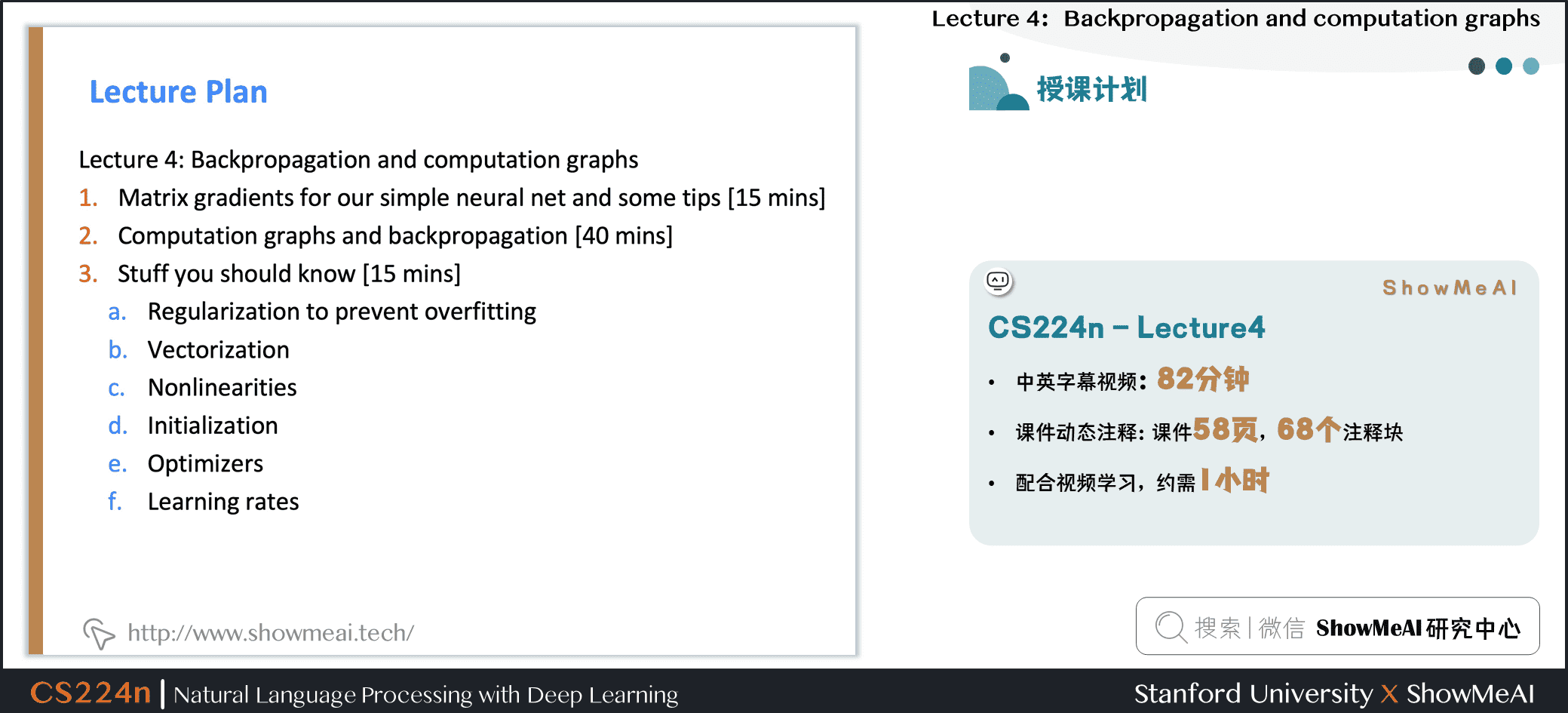• ① 简单神经网络的梯度矩阵与建议
• ② 计算图与反向传播
• ③ 神经网络训练实用知识技能 正则化（用于环节过拟合）
• 向量化
• 非线性表达能力
• 参数初始化
• 优化算法
• 学习率策略

# 1.简单神经网络的梯度矩阵与建议

## 1.1 权重矩阵的导数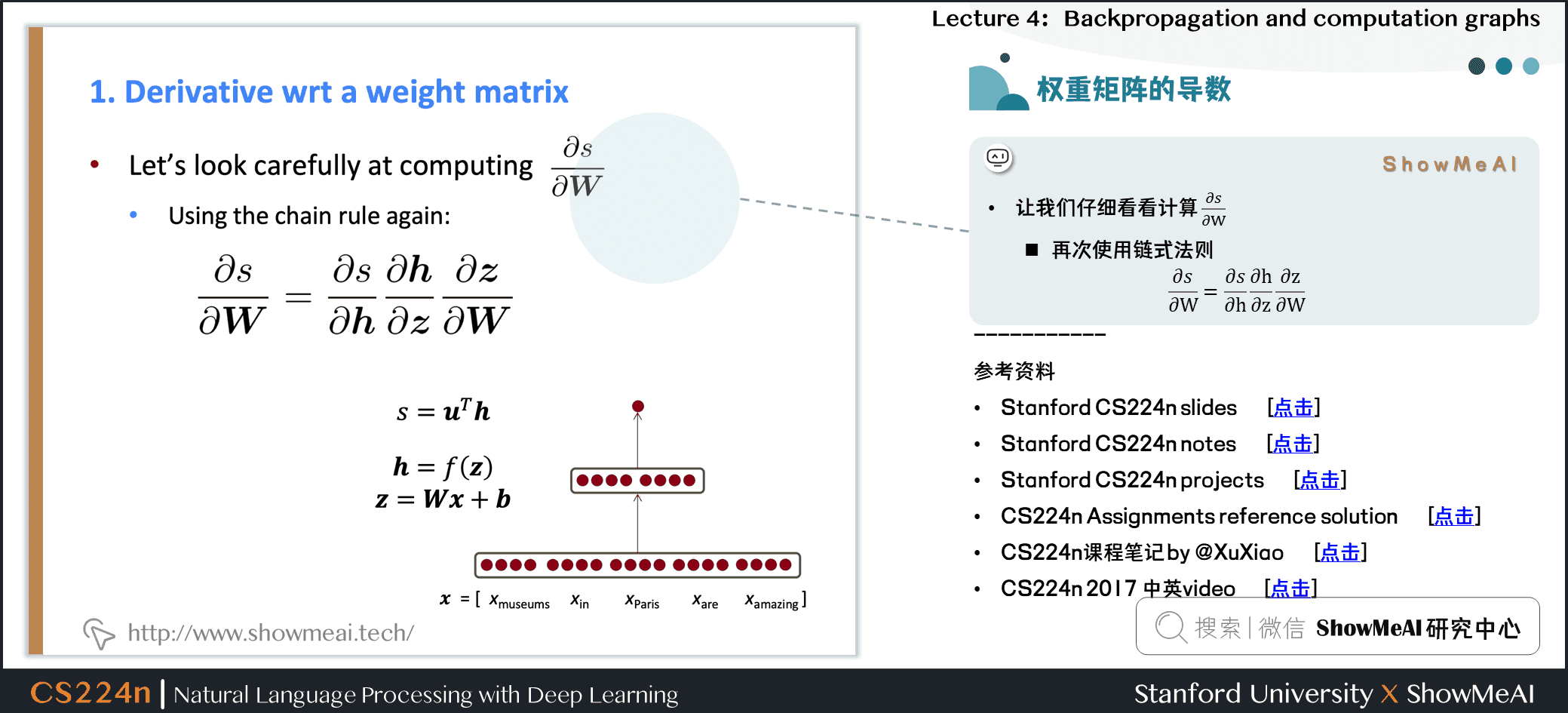• 让我们仔细看看计算 \frac{\partial s}{\partial W} 再次使用链式法则
\frac{\partial s}{\partial W}=\frac{\partial s}{\partial h} \frac{\partial h}{\partial z} \frac{\partial z}{\partial W} \begin{aligned} s &= u^T h \\ h &= f(z) \\ z &= Wx+b \end{aligned}

## 1.2 反向传播梯度求导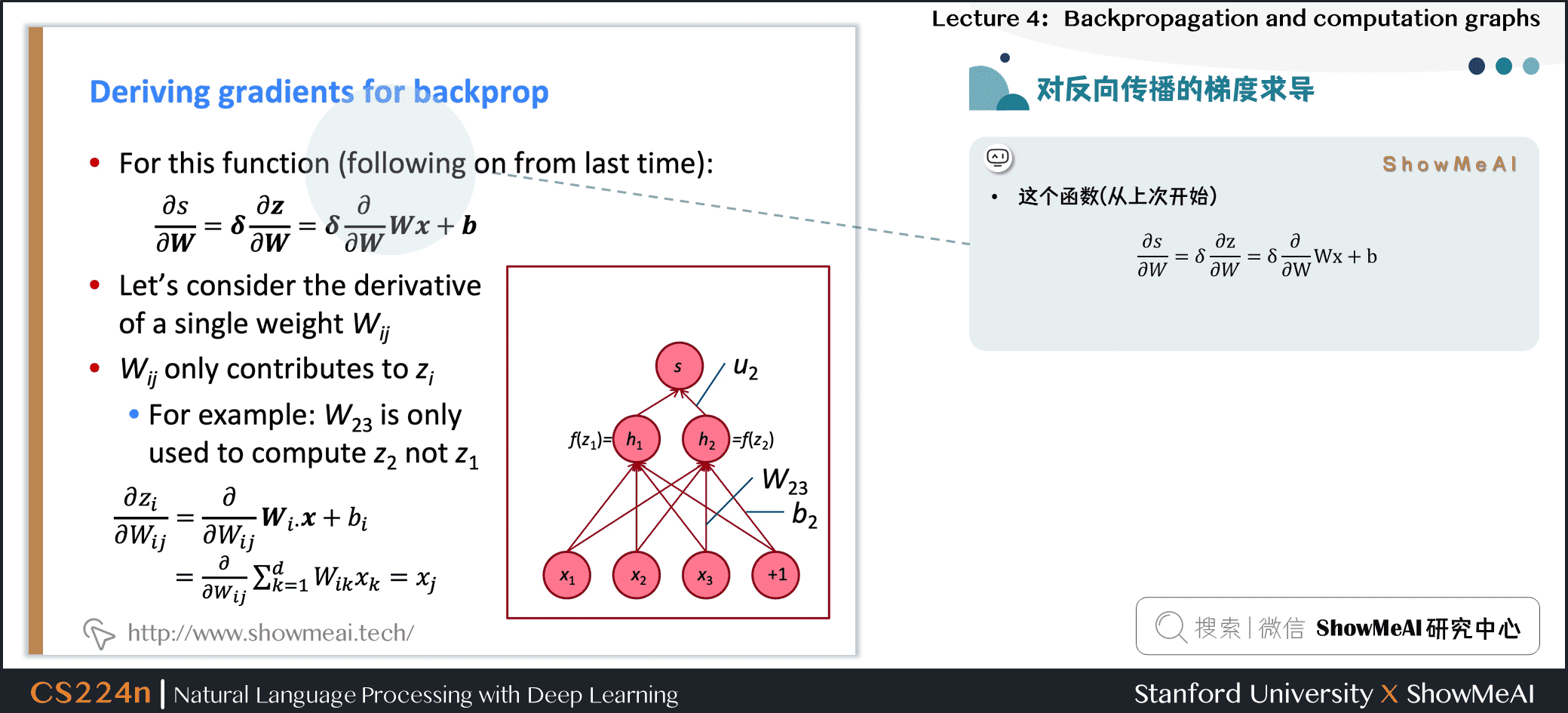• 这个函数(从上次开始)
\frac{\partial s}{\partial W}=\delta \frac{\partial z}{\partial W}=\delta \frac{\partial}{\partial W} Wx+b
• 考虑单个权重 W_{ij} 的导数
• W_{ij} 只对 z_i 有贡献 例如 W_{23} 只对 z_2 有贡献，对 z_1 没有贡献
\begin{aligned} \frac{\partial z_{i}}{\partial W_{i j}} &=\frac{\partial}{\partial W_{i j}} W_{i \cdot} x+b_{i} \\ &=\frac{\partial}{\partial W_{i j}} \sum_{k=1}^{d} W_{i k} x_{k}=x_{j} \end{aligned}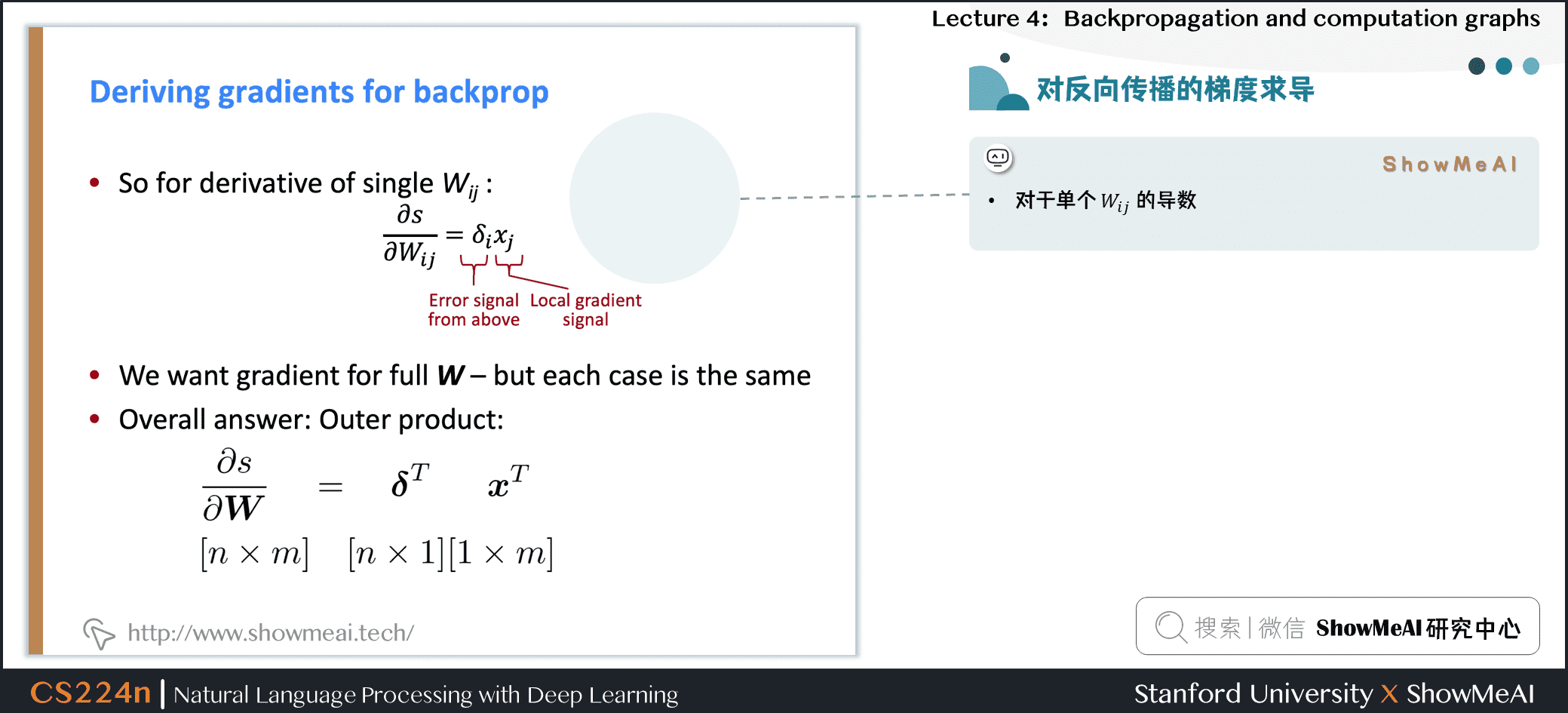• 对于单个 W_{ij} 的导数：
\frac{\partial s}{\partial W_{i j}} = \delta_i x_j
• 我们想要整个 W 的梯度，但是每种情况都是一样的
• 解决方案：外积
\begin{aligned} \frac{\partial s}{\partial {W}}&=\delta ^{T} x^{T}\\ [n \times m] &= [n \times 1][1 \times m] \end{aligned}

## 1.3 梯度求导：技巧与建议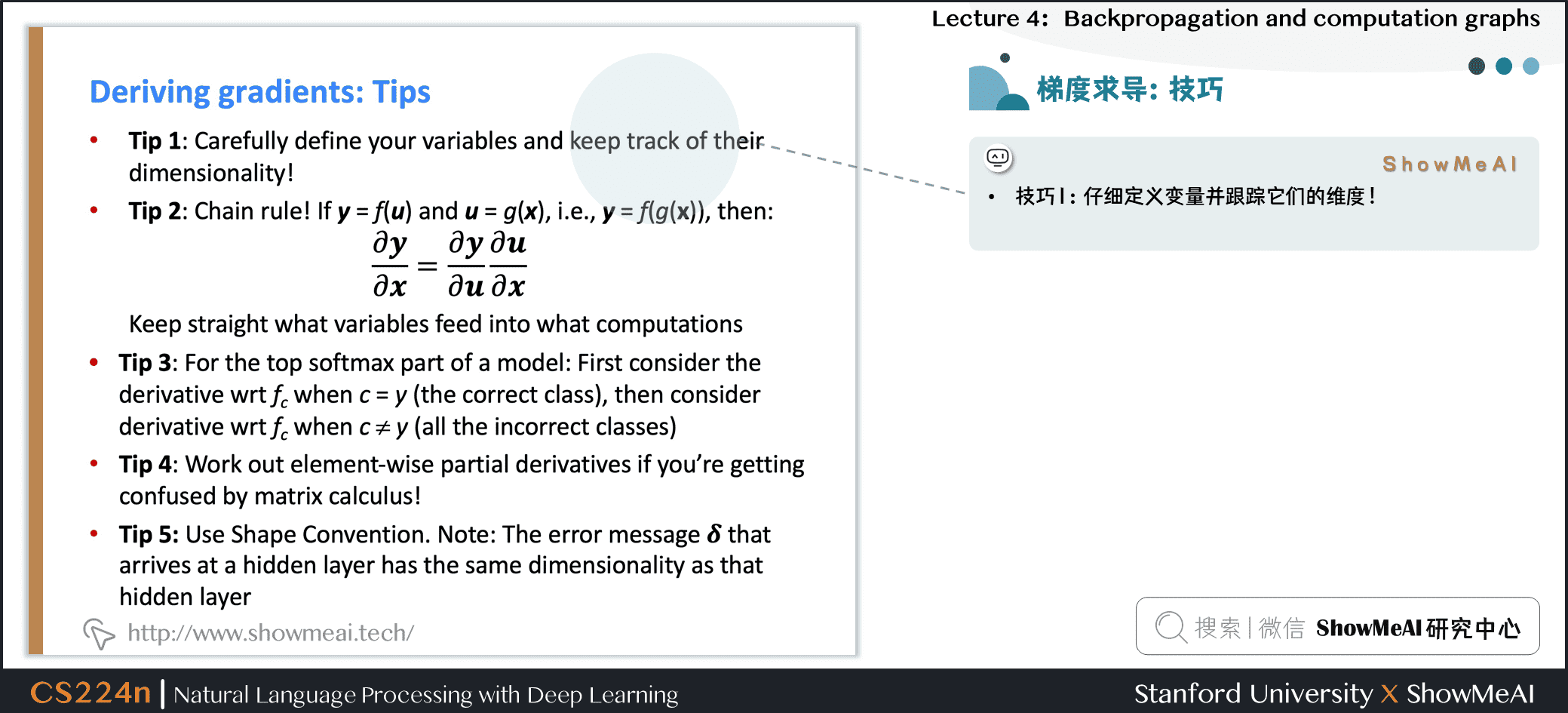• 技巧1：仔细定义变量并关注它们的维度！

• 技巧2：链式法则！如果 y = f(u) , u = g(x)，即 y = f(g(x)) 则

\frac{\partial {y}}{\partial {x}}=\frac{\partial {y}}{\partial {u}} \frac{\partial {u}}{\partial {x}}
• 提示3：模型的最上面的softmax部分：首先考虑当 c = y (正确的类)的导数 f_c，然后再考虑当 c \neq y (所有不正确的类)的导数 f_c

• 技巧4：如果你被矩阵微积分搞糊涂了，请计算逐个元素的偏导数！

• 技巧5：使用形状约定。注意：到达隐藏层的错误消息 \delta 具有与该隐藏层相同的维度

## 1.4 为窗口模型推导梯度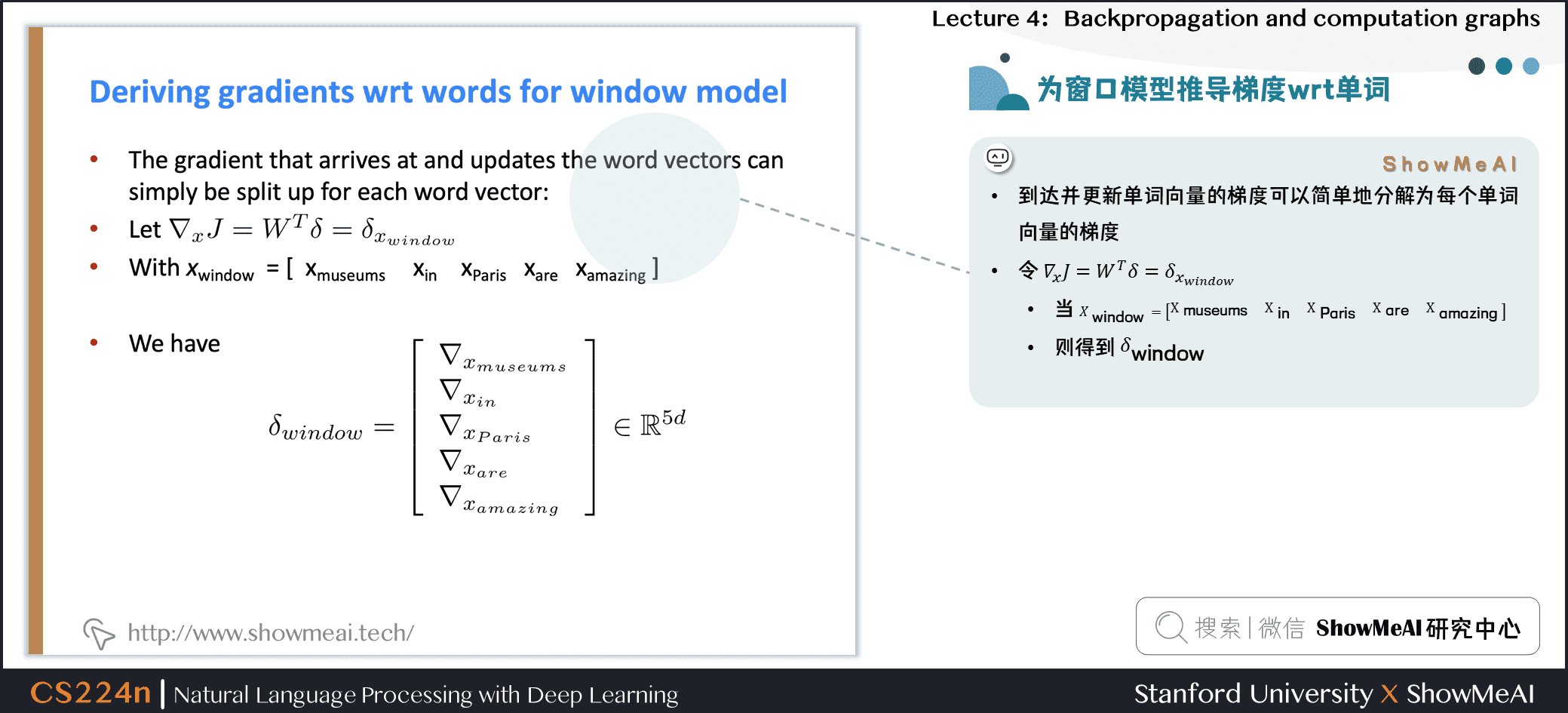• 到达并更新单词向量的梯度可以简单地分解为每个单词向量的梯度

• 令 \nabla_{x} J=W^{T} \delta=\delta_{x_{w i n d o w}}

• 则得到

\begin{aligned} \delta_{window}=\left[\begin{array}{c}{\nabla_{x_{\text {museums}}}} \\ {\nabla_{x_{i n}}} \\ {\nabla_{x_{\text {Pare}}}} \\ {\nabla_{x_{\text {are}}}} \\ {\nabla_{x_{\text {amazing}}}}\end{array}\right] \in \mathbb{R}^{5 d} \end{aligned}
• 我们将根据梯度逐个更新对应的词向量矩阵中的词向量，所以实际上是对词向量矩阵的更新是非常稀疏的

## 1.5 在窗口模型中更新单词梯度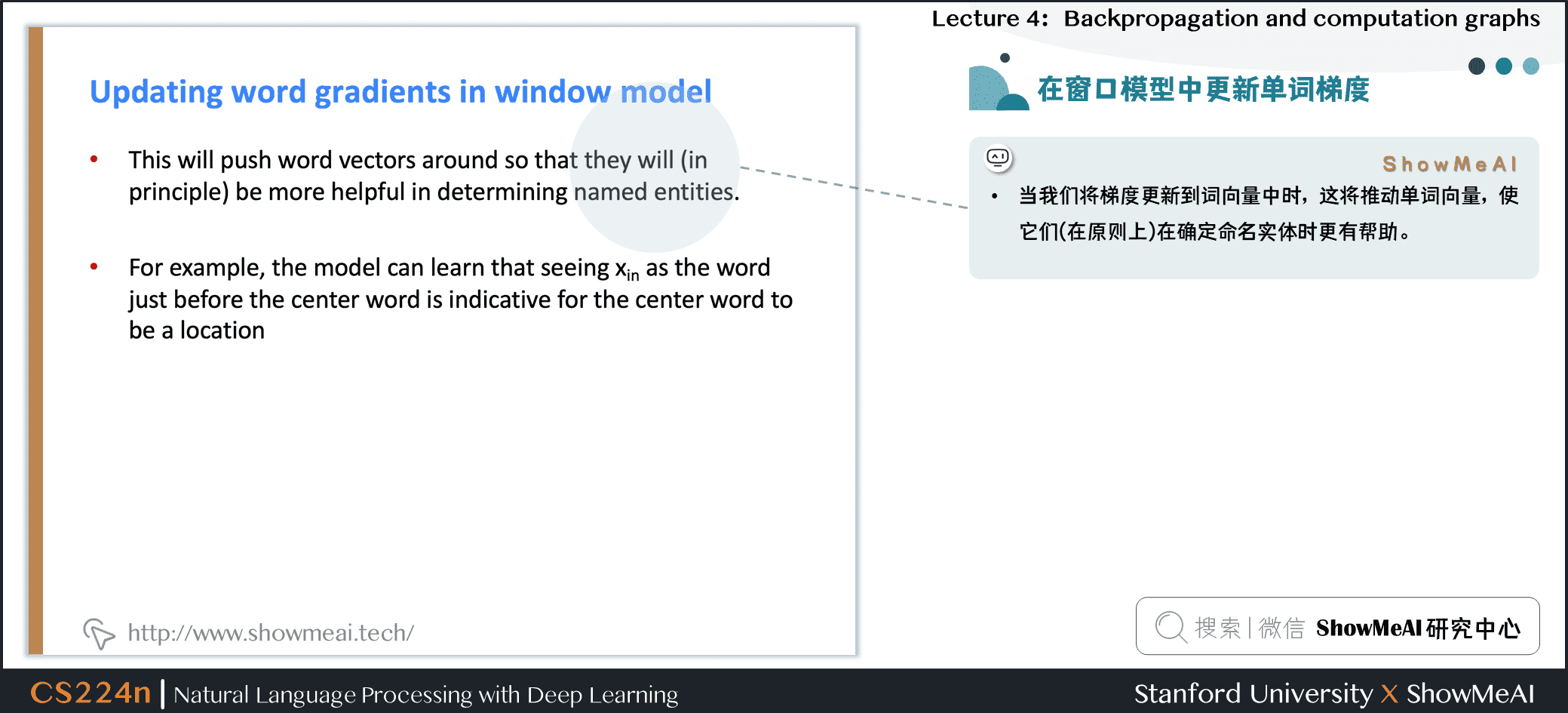• 当我们将梯度更新到词向量中时，这将更新单词向量，使它们(理论上)在确定命名实体时更有帮助。
• 例如，模型可以了解到，当看到 x_{in} 是中心词之前的单词时，指示中心词是一个 Location

## 1.6 重新训练词向量时的陷阱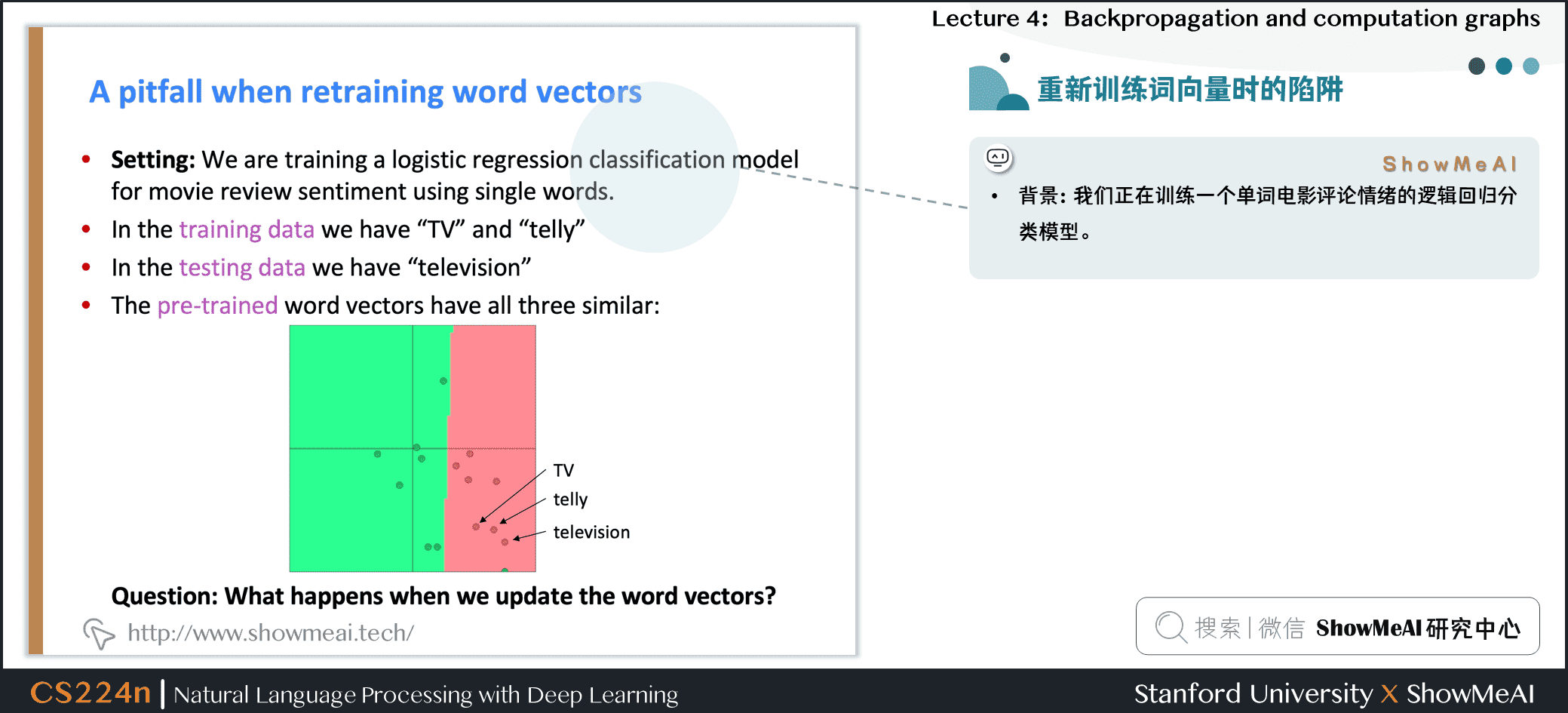• 训练数据中，我们有“TV”和“telly”
• 测试数据中我们有“television””
• 预训练的单词向量有三个相似之处：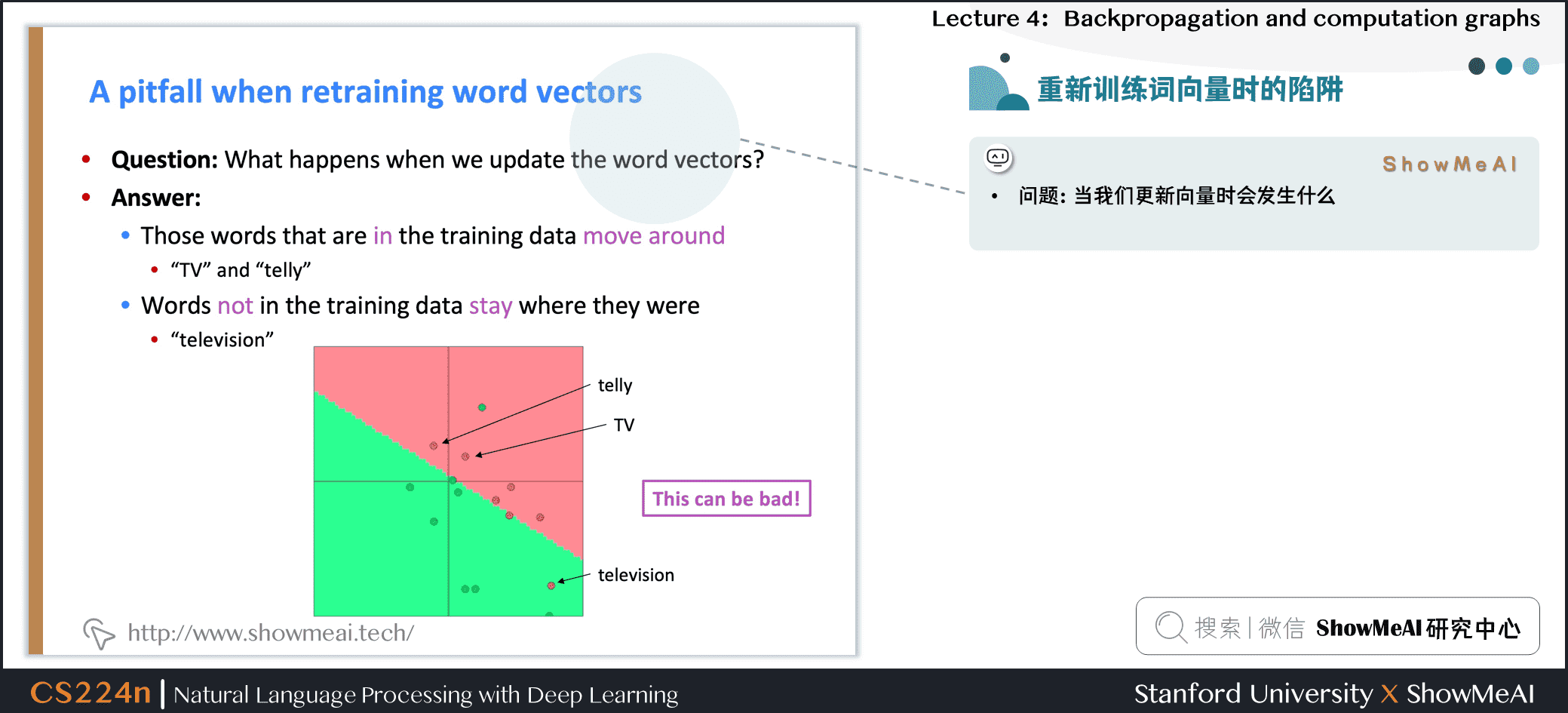• 问题：当我们更新向量时会发生什么

• 回答

那些在训练数据中出现的单词会四处移动 “TV”和“telly”
• 没有包含在训练数据中的词汇保持原样
“television”

## 1.7 关于再训练的建议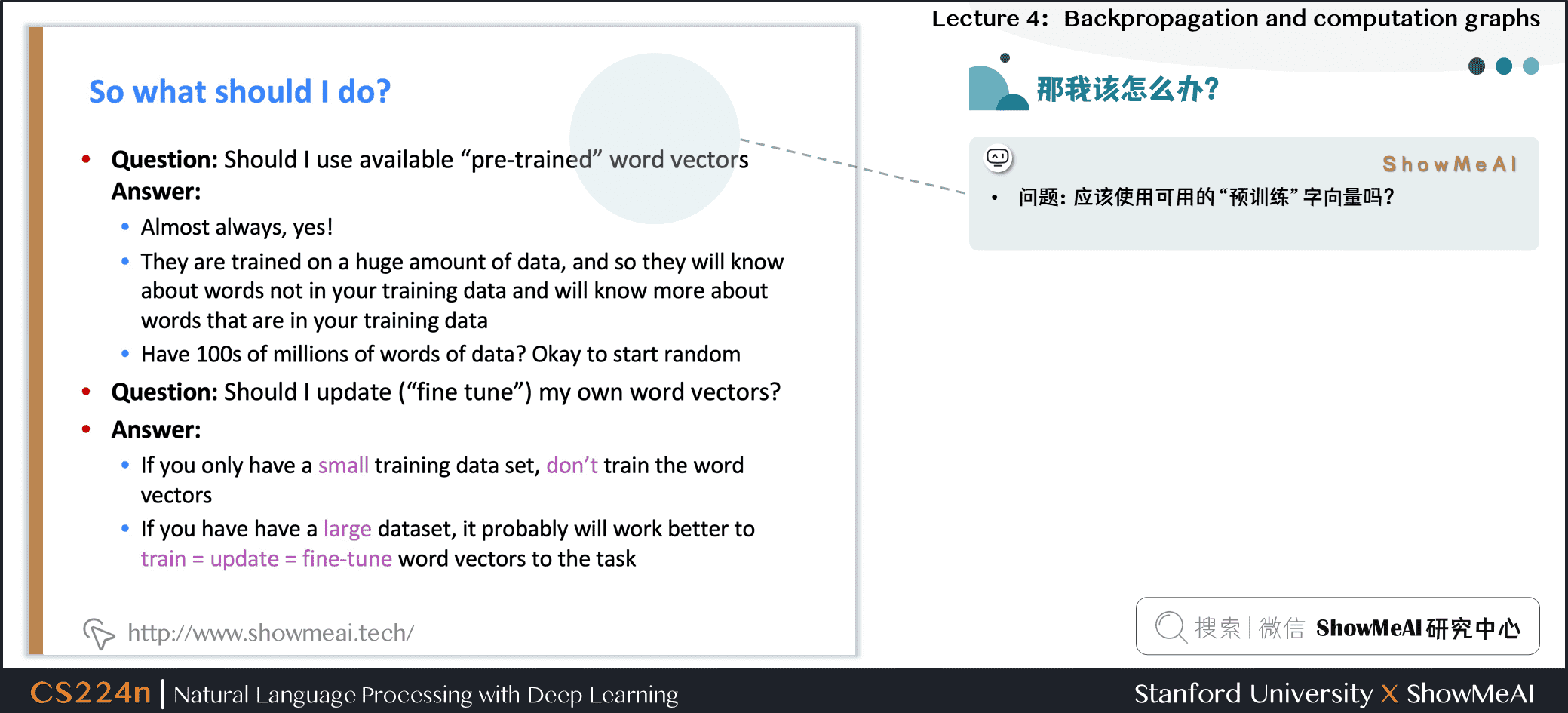• 问题：应该使用可用的“预训练”词向量吗？

• 回答

几乎总是「应该用」
• 他们接受了大量的数据训练，所以他们会知道训练数据中没有的单词，也会知道更多关于训练数据中的单词
• 拥有上亿的数据语料吗？那可以随机初始化开始训练
• 问题：我应该更新(“fine tune”)我自己的单词向量吗？

• 回答

如果你只有一个小的训练数据集，不要对预训练词向量做再训练
• 如果您有一个大型数据集，那么基于任务训练更新词向量（ train = update = fine-tune ）效果会更好

# 2.计算图与反向传播

## 2.1 反向传播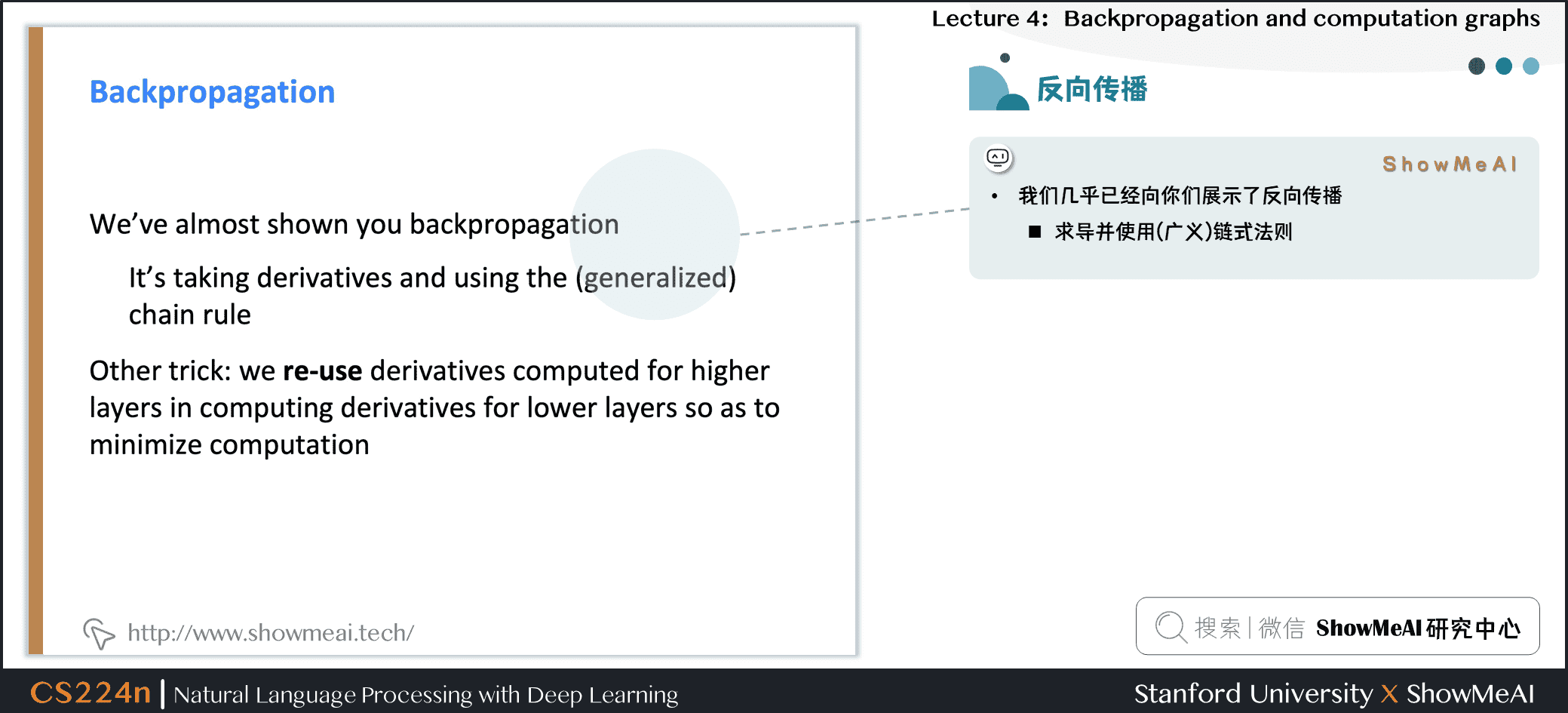• 我们几乎已经向你们展示了反向传播

求导并使用(广义)链式法则
• 另一个技巧：在计算较低层的导数时，我们重用对较深层计算的导数，以减小计算量

• ## 2.2 计算图和反向传播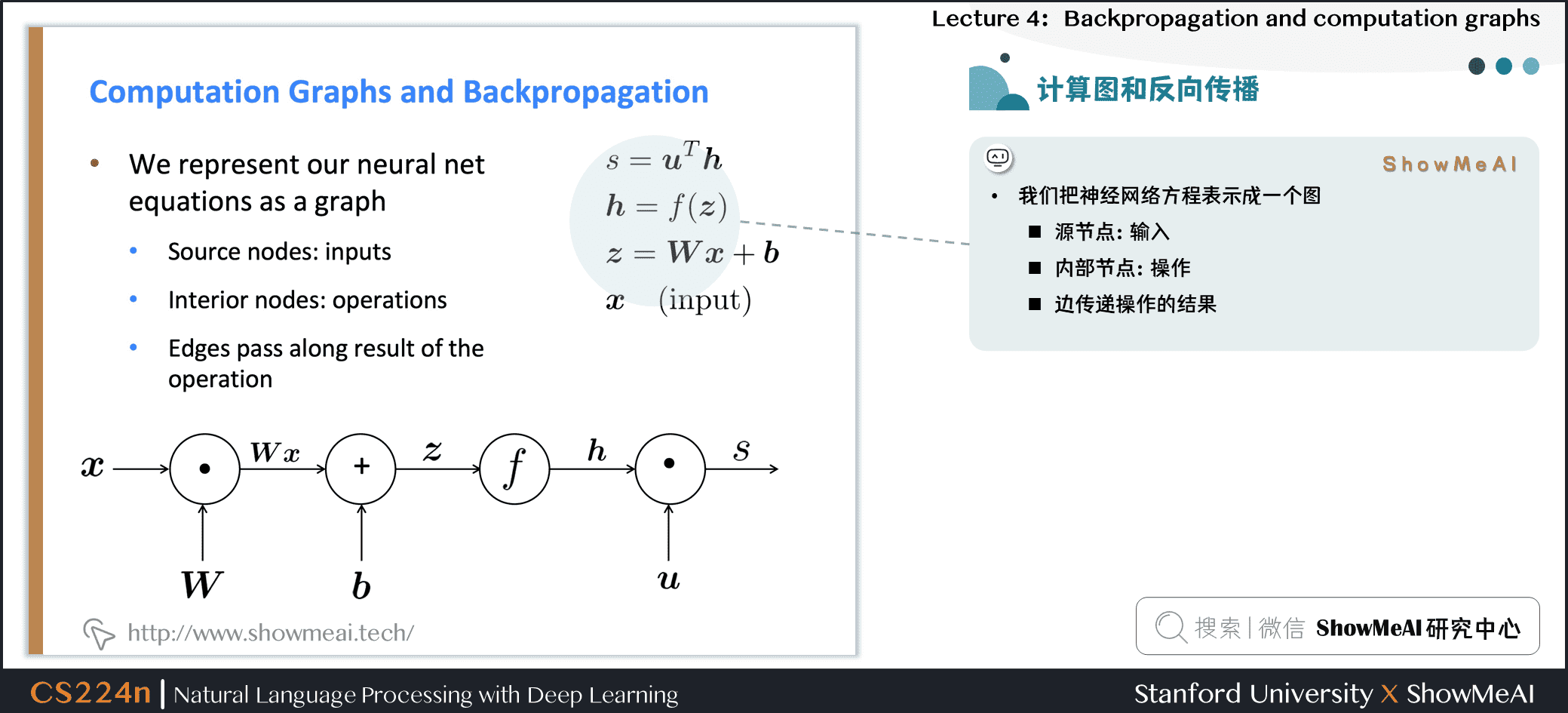• 我们把神经网络方程表示成一个图 源节点：输入
• 内部节点：操作
• 边传递操作的结果
\begin{aligned} s &= u^Th \\ h &= f(z) \\ z &= Wx+b \\ x & \quad (input) \end{aligned}

Forward Propagation：前向传播 Back Propagation：沿着边回传梯度

## 2.3 反向传播：单神经元视角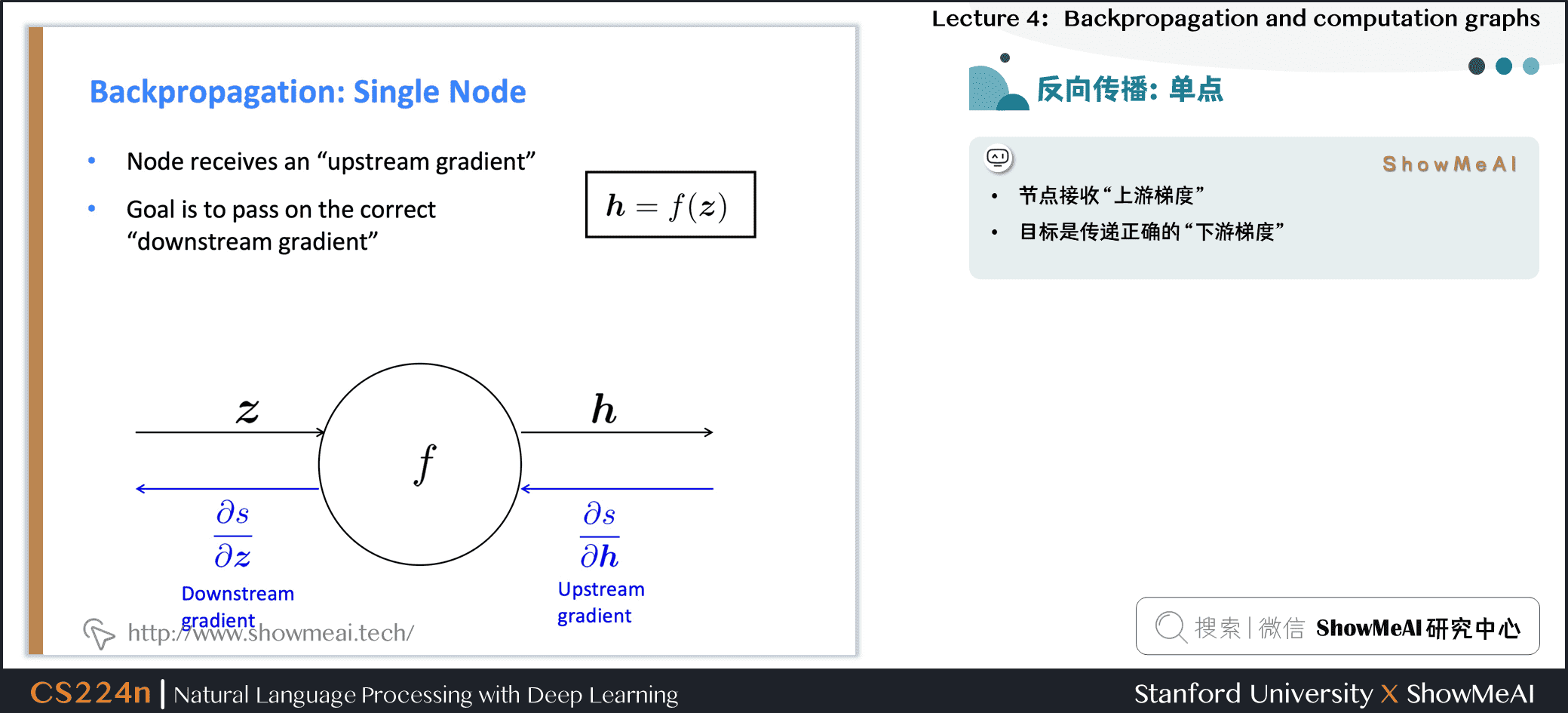• 节点接收“上游梯度”

目标是传递正确的“下游梯度”

它输出的梯度是与它的输入有关

n它输出的梯度是与它的输入有关

它输出的梯度是与它的输入有关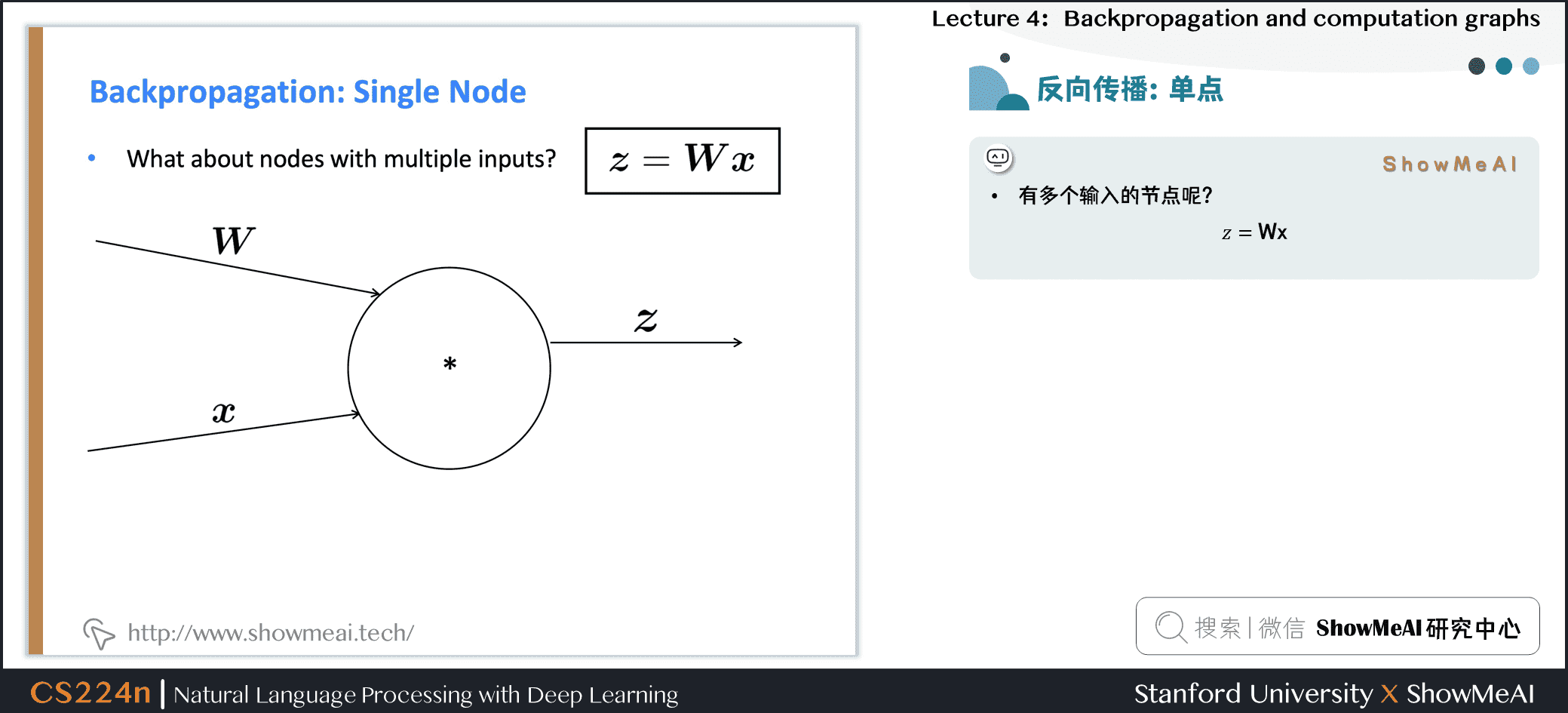• 有多个输入的节点呢？z=Wx
• 多个输入 → 多个局部梯度

## 2.4 反向传播计算图示例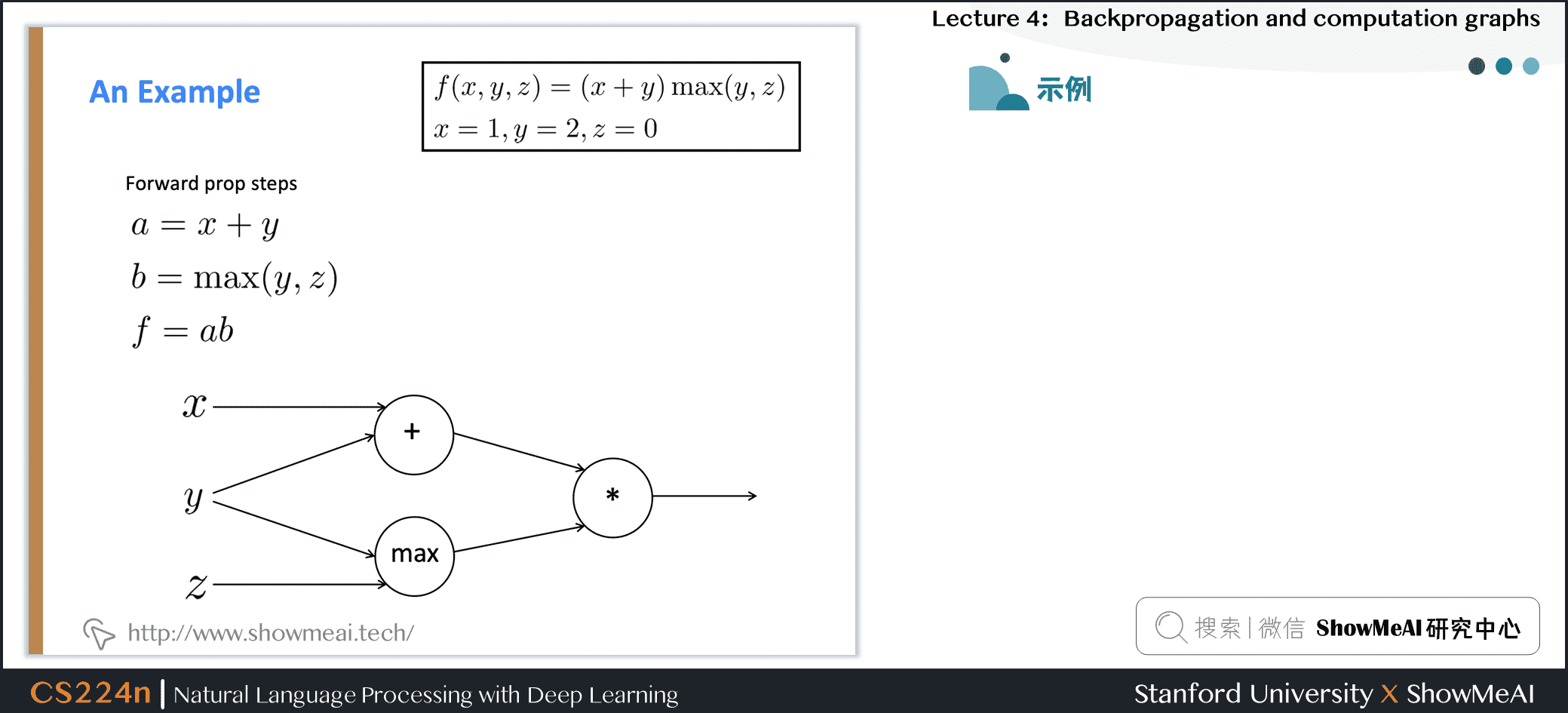## 2.5 求和形态的梯度计算

上图中的 \frac{\partial f}{\partial y} 的梯度的计算

\begin{aligned} a &= x + y \\ b &= max(y,z)\\ f &= ab \end{aligned} \frac{\partial f}{\partial y} = \frac{\partial f}{\partial a}\frac{\partial a}{\partial y} + \frac{\partial f}{\partial b}\frac{\partial b}{\partial y}

## 2.6 直挂理解神经元的梯度传递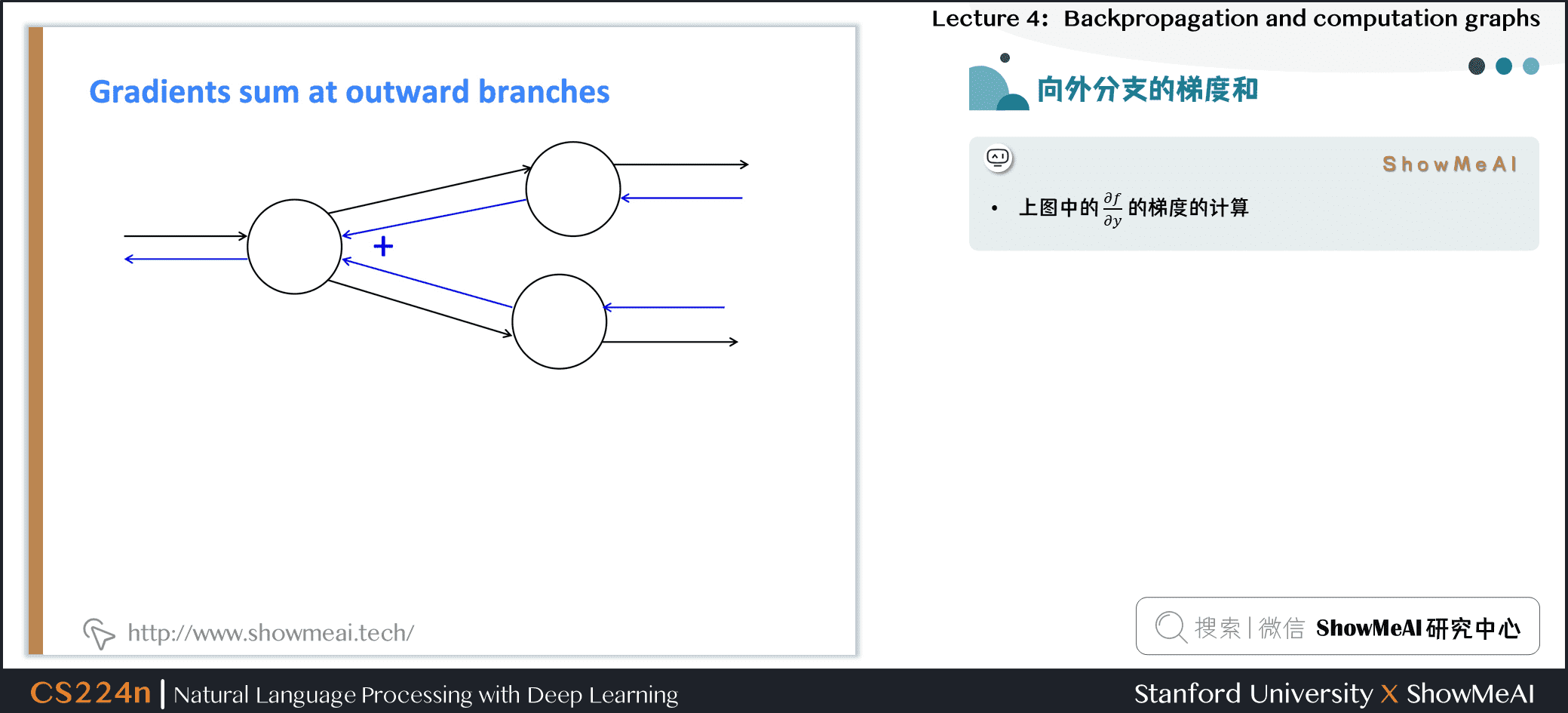• + “分发” 上游梯度
• max “路由” 上游梯度，将梯度发送到最大的方向
• \ast “切换”上游梯度

## 2.7 同步计算所有梯度以提速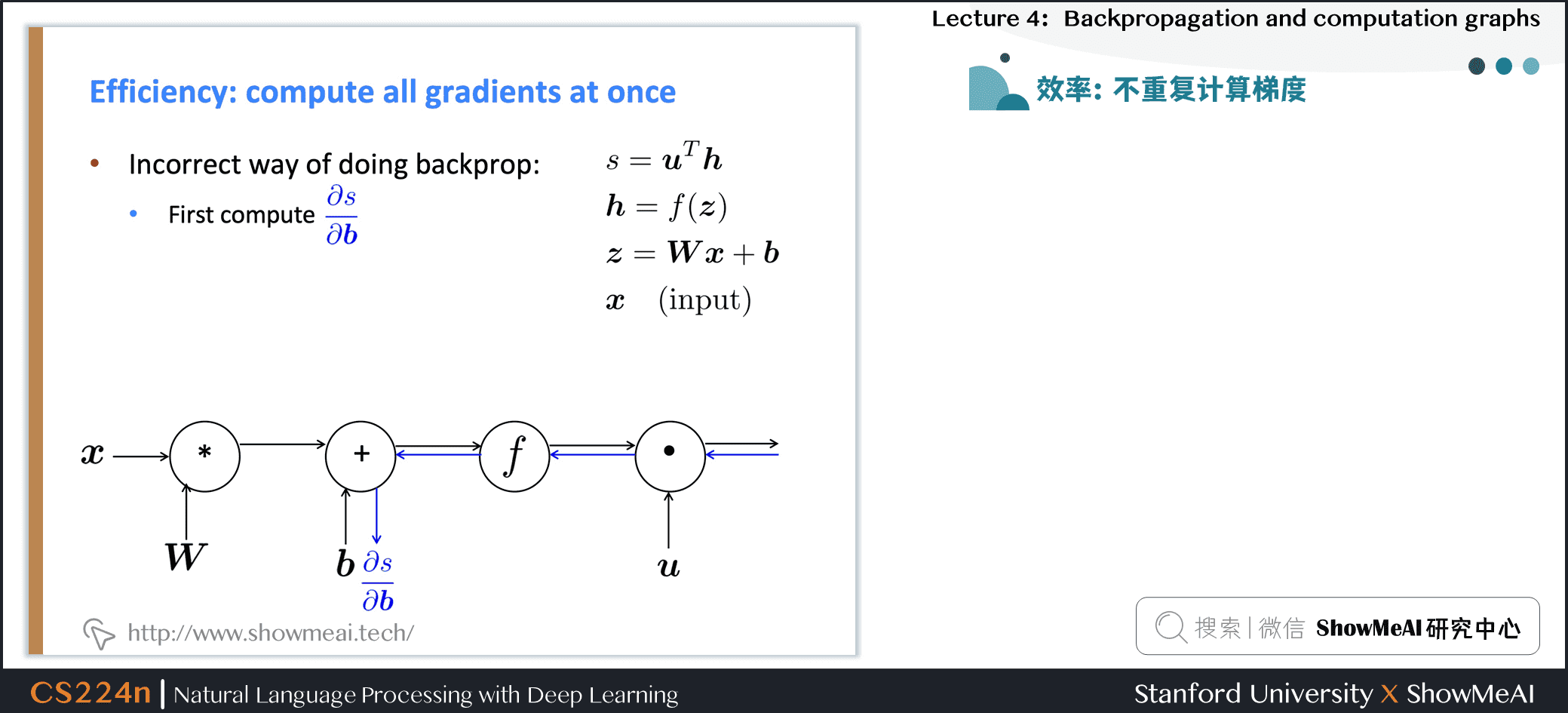• 错误的反向传播计算方式

先计算$b$的偏导
• 接着计算$W$的偏导
• 重复计算！
• 正确的反向传播计算方式

一次性计算所有偏导
• 类似手动计算梯度时的方式

## 2.8 一般计算图中的反向传播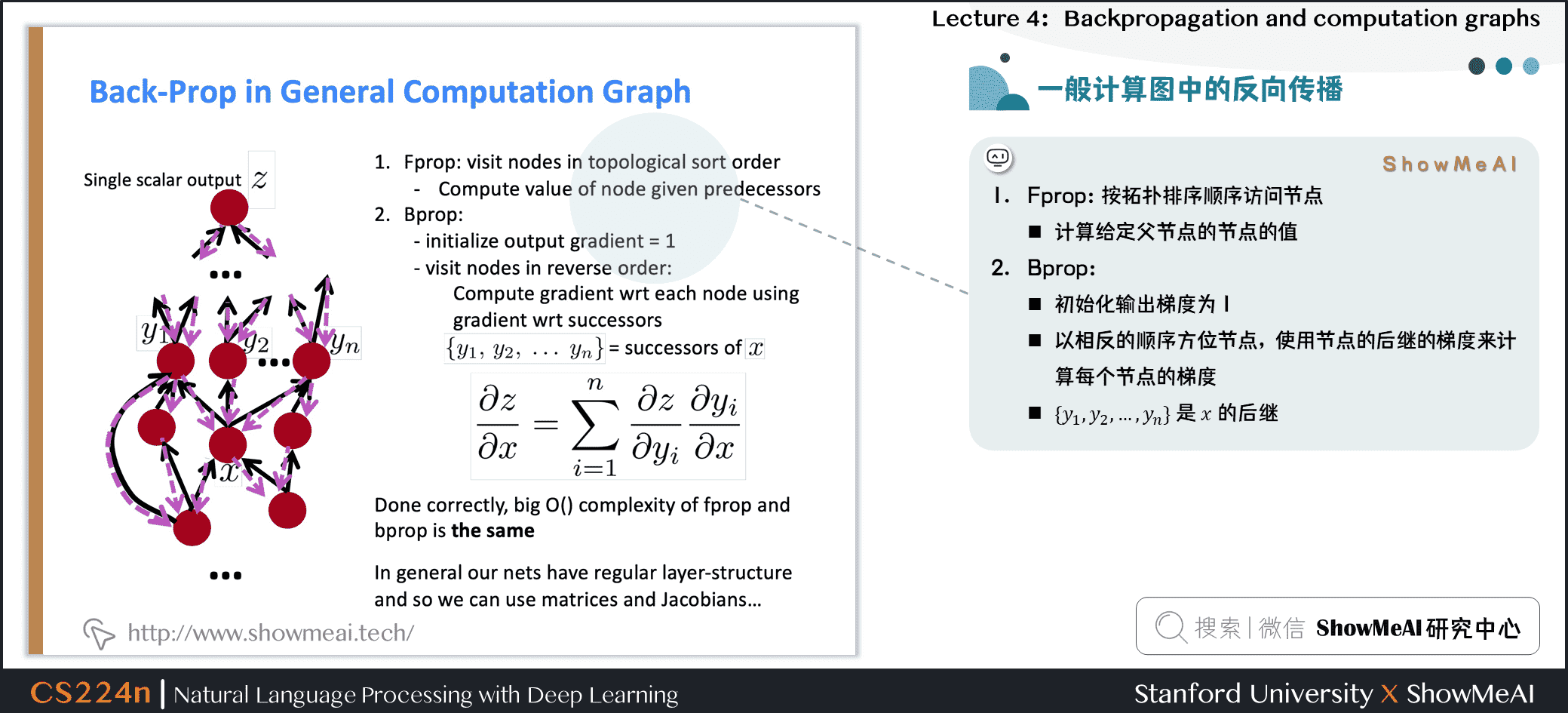• Fprop：按拓扑排序顺序访问节点

计算给定父节点的节点的值
• Bprop

初始化输出梯度为 1
• 以相反的顺序方位节点，使用节点的后继的梯度来计算每个节点的梯度
• \{y_1,y_2,\cdots,y_n\} 是 x 的后继
\frac{\partial z}{\partial x} = \sum_{i=1}^n \frac{\partial z}{\partial y_i}\frac{\partial y_i}{\partial x}
• 正确地说，Fprop 和 Bprop 的计算复杂度是一样的
• 一般来说，我们的网络有固定的层结构，所以我们可以使用矩阵和雅可比矩阵

## 2.9 自动微分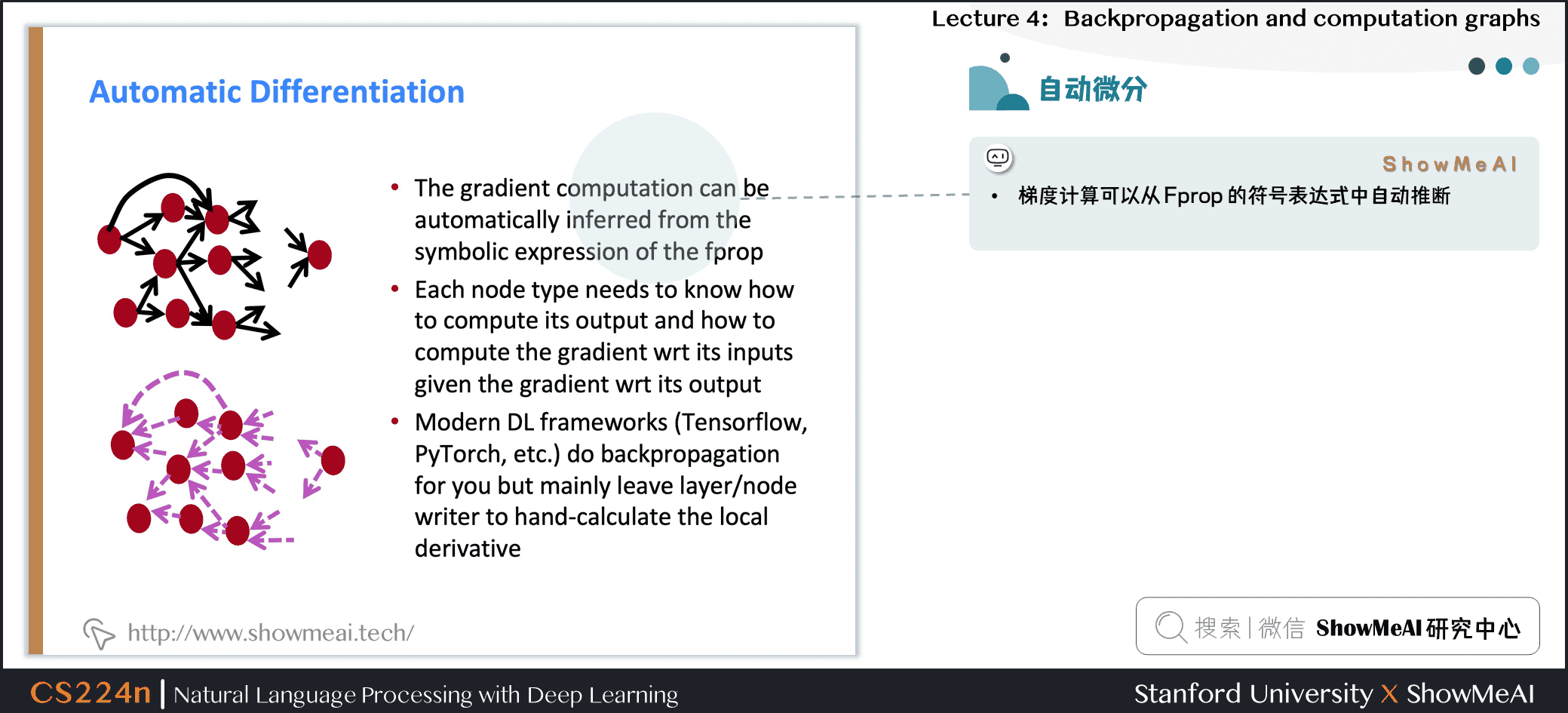• 梯度计算可以从 Fprop 的符号表达式中自动推断
• 每个节点类型需要知道如何计算其输出，以及如何在给定其输出的梯度后计算其输入的梯度
• 现代DL框架(Tensorflow, Pytoch)为您做反向传播，但主要是令作者手工计算层/节点的局部导数

## 2.10 反向传播的实现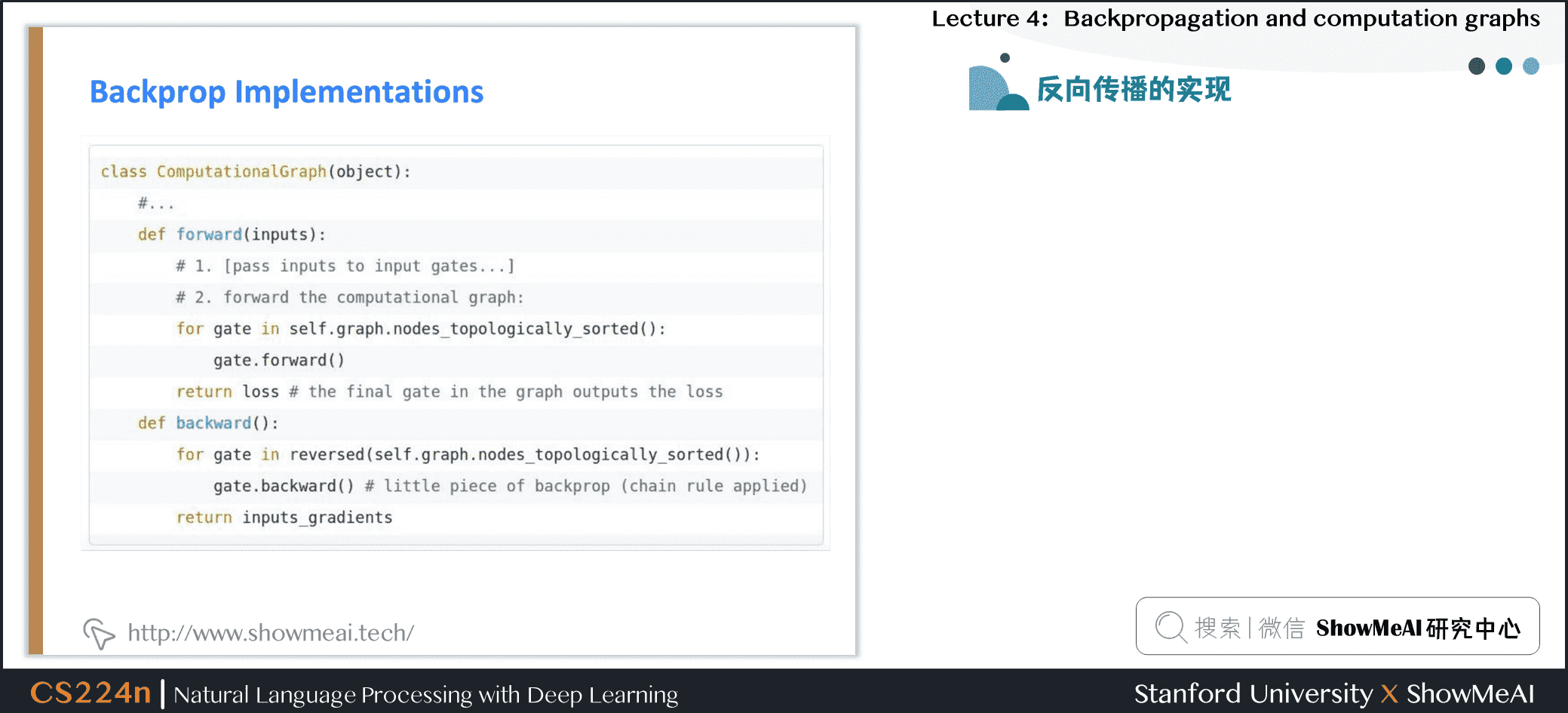为了计算反向传播，我们需要在前向传播时存储一些变量的值

## 2.11 实现：前向/反向API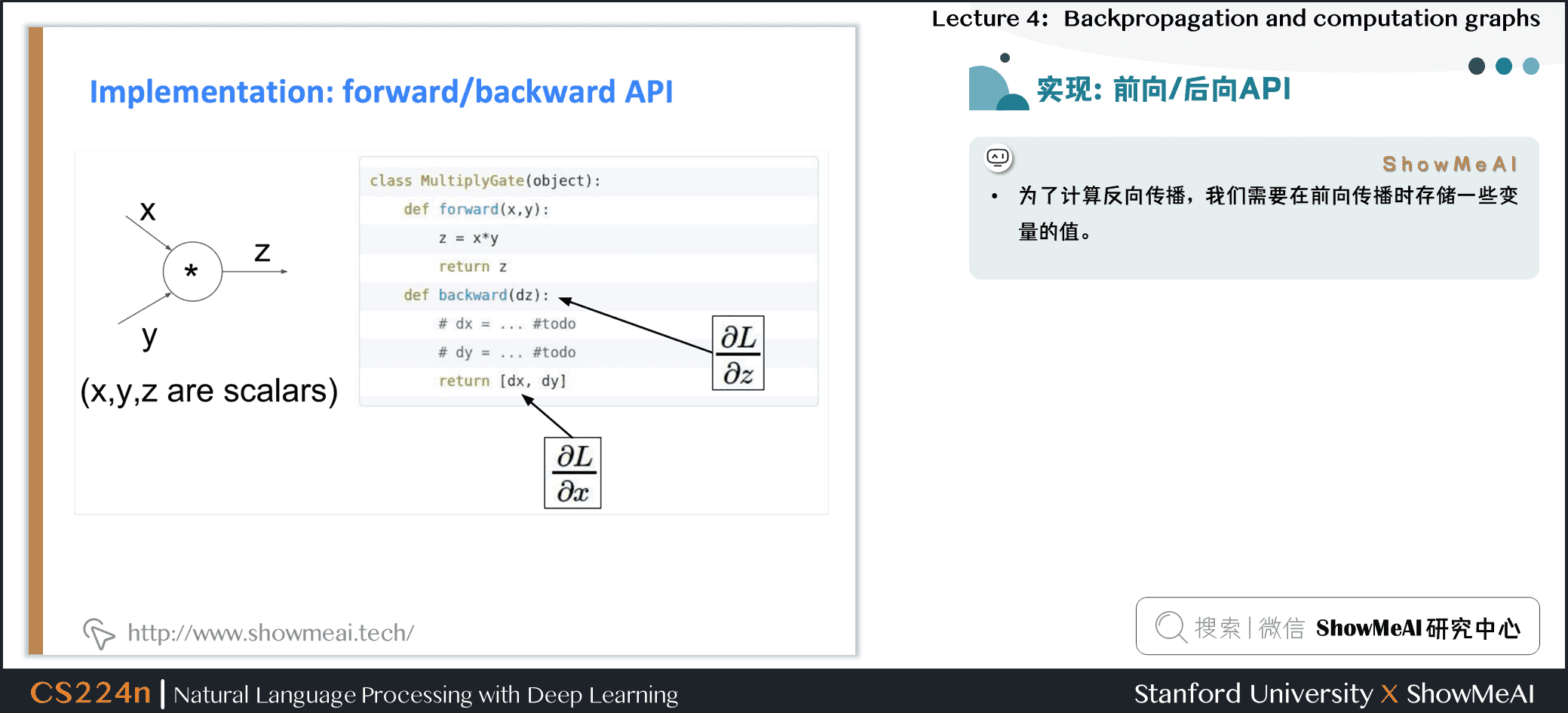为了计算反向传播，我们需要在前向传播时存储一些变量的值

## 2.12 梯度检查：数值梯度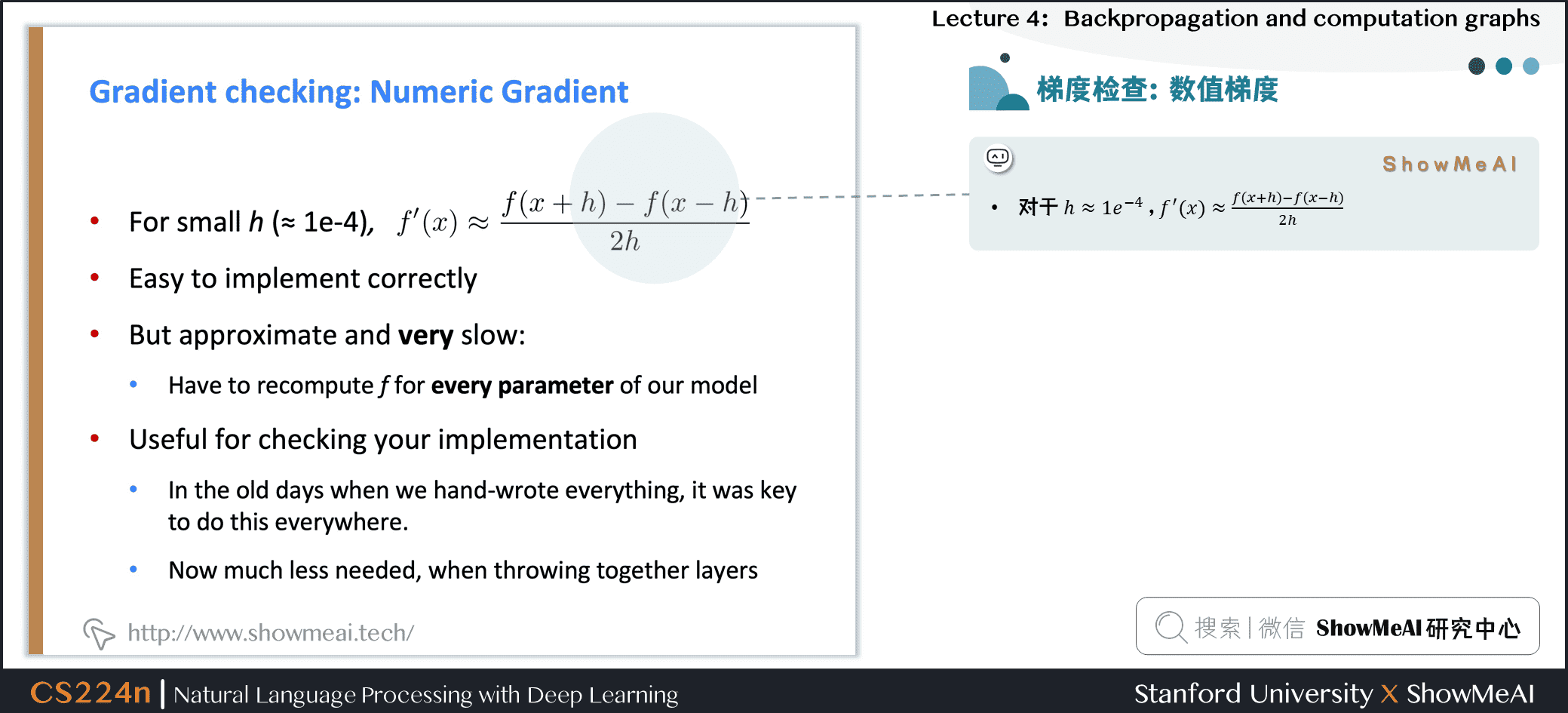• 对于 h \approx 1e^{-4} , f^{\prime}(x) \approx \frac{f(x+h)-f(x-h)}{2 h}

• 易于正确实现

• 但近似且非常缓慢

必须对模型的每个参数重新计算 f
• 用于检查您的实现

在过去我们手写所有东西的时候，在任何地方都这样做是关键。
• 现在，当把图层放在一起时，就不需要那么多了

## 2.13 总结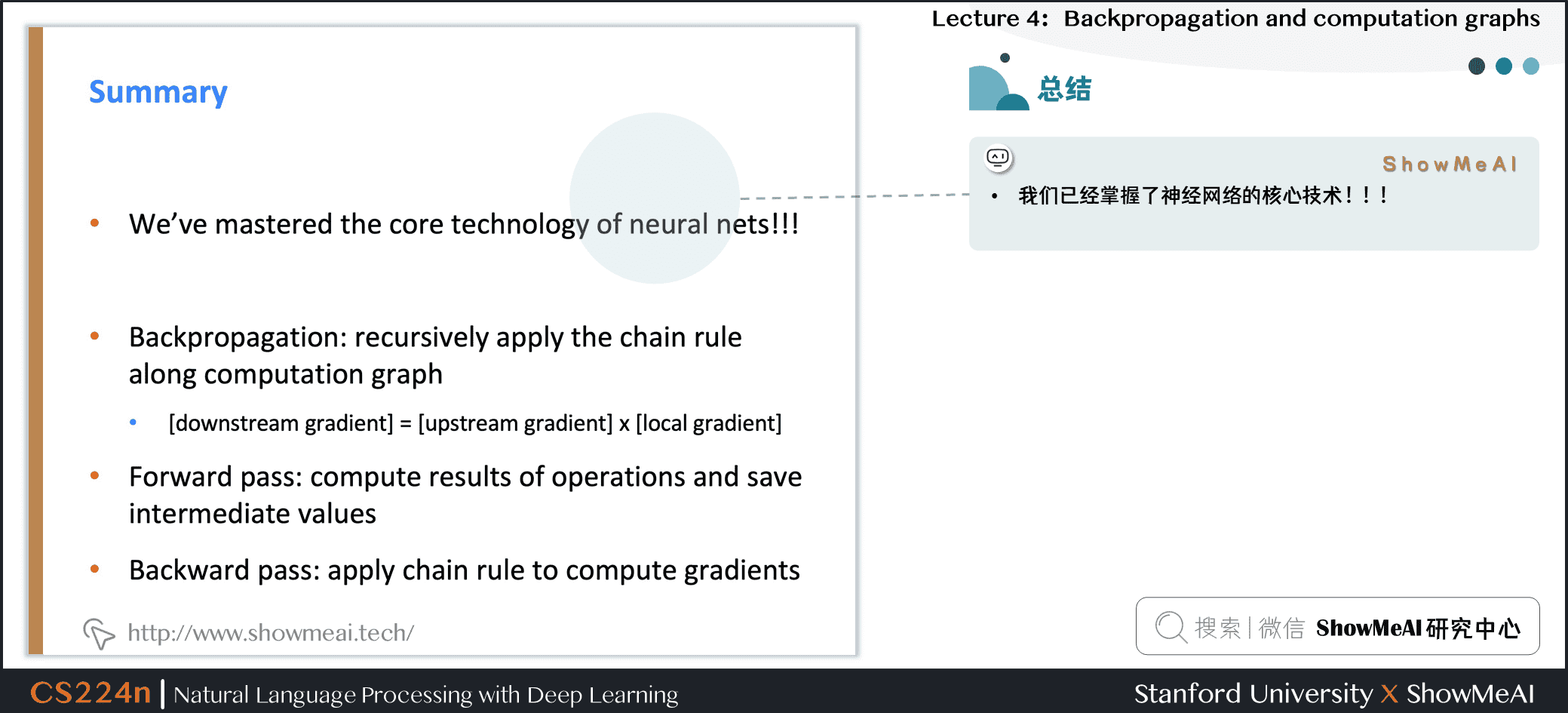• 我们已经掌握了神经网络的核心技术
• 前向传递：计算操作结果并保存中间值
• 反向传递：应用链式法则计算梯度
• ## 2.14 为什么要学习梯度的所有细节？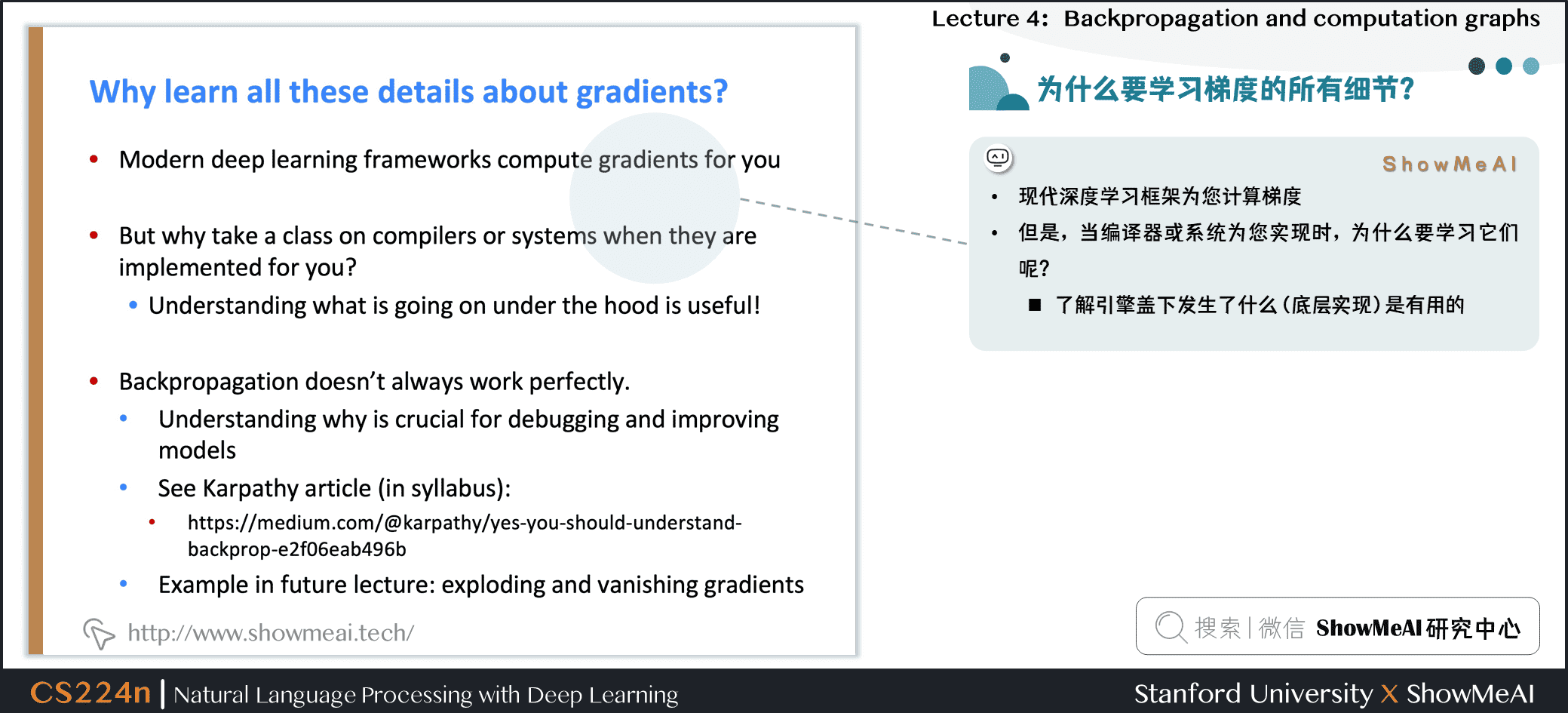• 现代深度学习框架为您计算梯度

• 但是，当编译器或系统为您实现时，为什么要学习它们呢？

了解底层原理是有帮助的
• 反向传播并不总是完美地工作

理解为什么对调试和改进模型至关重要
• 参见 Karpathy文章
• 未来课程的例子:爆炸和消失的梯度

• # 3.神经网络训练实用知识技能

## 3.1 模型正则化防止过拟合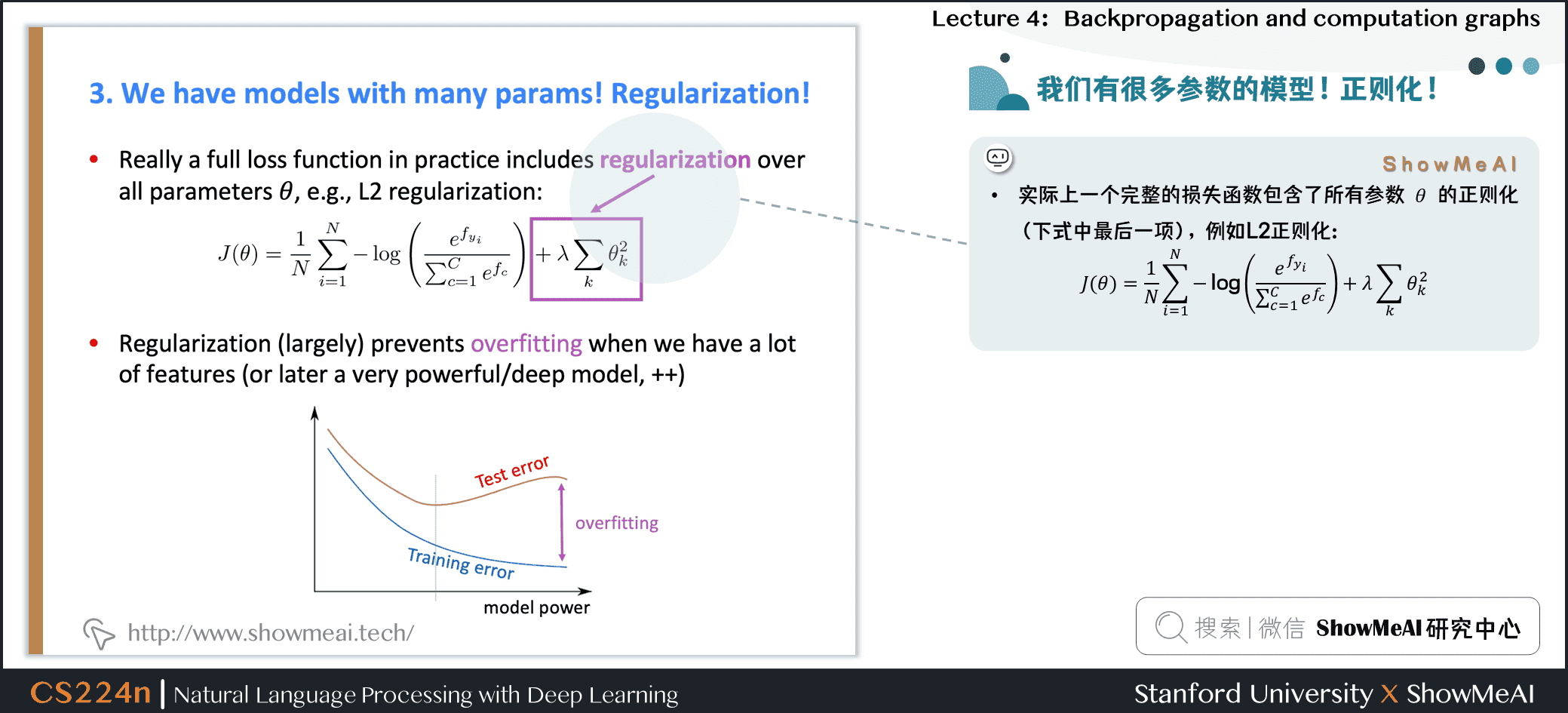• 实际上一个完整的损失函数包含了所有参数$\theta$的正则化（下式中最后一项），例如L2正则化：
J(\theta)=\frac{1}{N} \sum_{i=1}^{N}-\log (\frac{e^{f_{y_{i}}}}{\sum_{c=1}^{C} e^{f_{c}}})+\lambda \sum_{k} \theta_{k}^{2}
• 正则化(在很大程度上)可以防止在我们有很多特征时过拟合(或者是一个非常强大/深层的模型等等)

## 3.2 向量化形态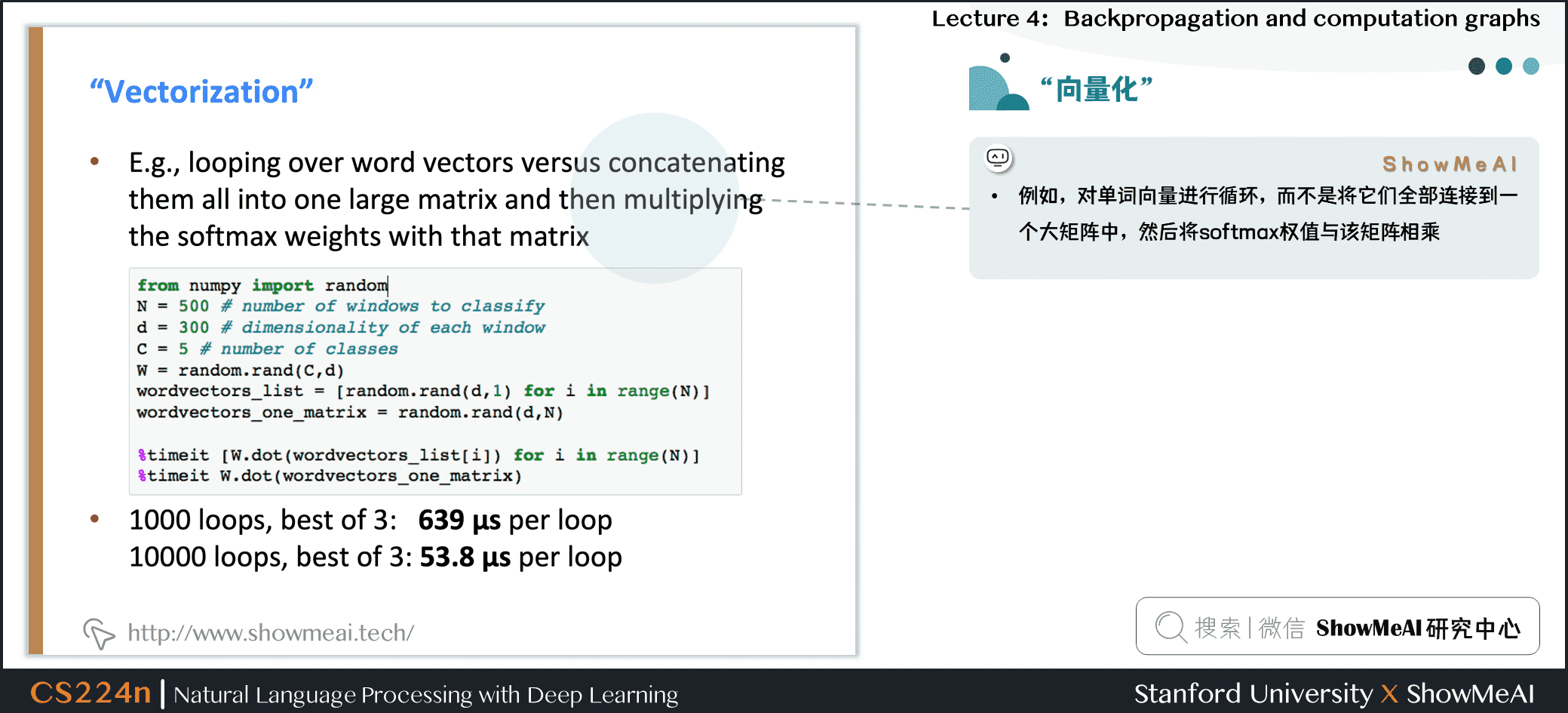• 例如，对单词向量进行循环，而不是将它们全部连接到一个大矩阵中，然后将softmax权值与该矩阵相乘

1000 loops, best of 3: 639 μs per loop
• 10000 loops, best of 3: 53.8 μs per loop
• (10x)更快的方法是使用 C \times N 矩阵

• 总是尝试使用向量和矩阵，而不是循环

• 你也应该快速测试你的代码

• 简单来说：矩阵向量化的方式太棒了

• ## 3.3 非线性：常规激活函数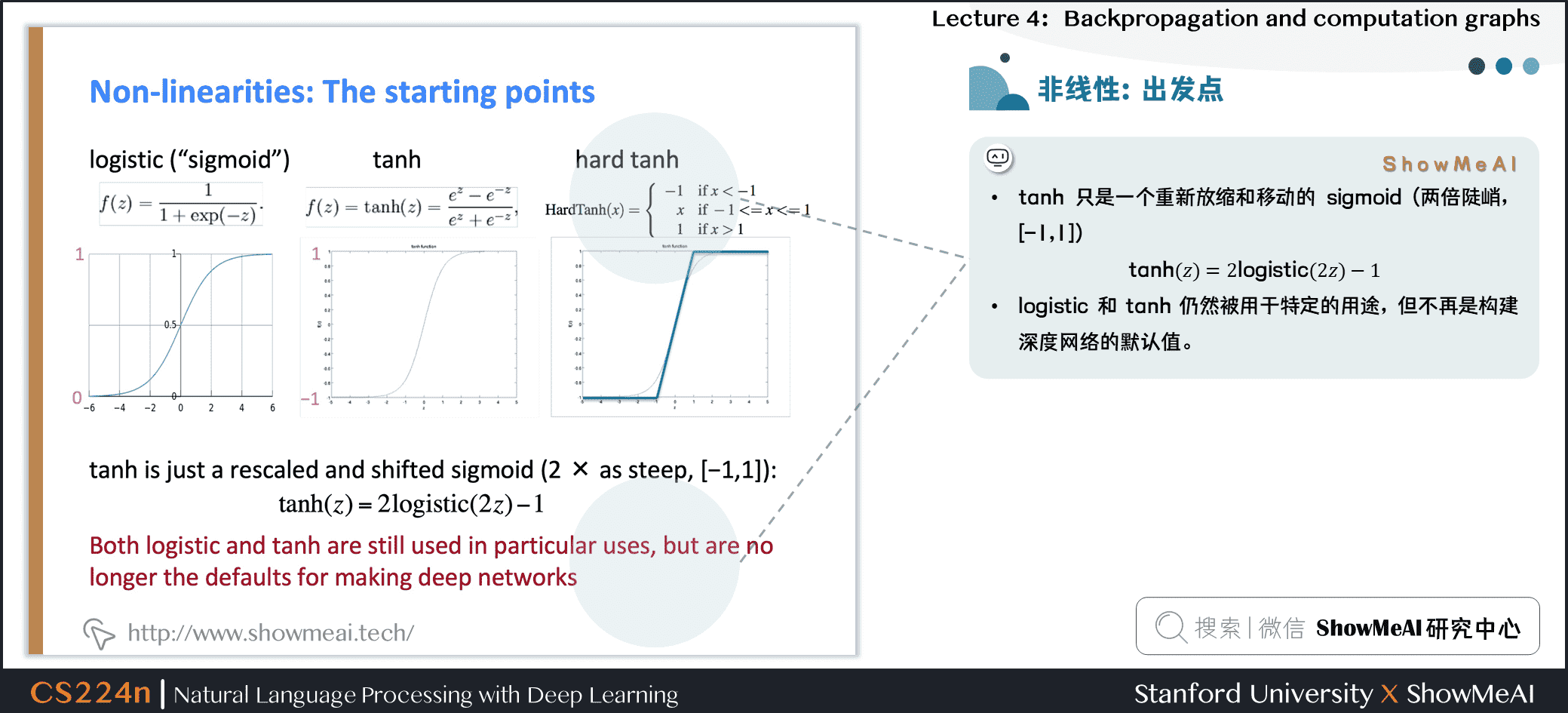tanh 只是一个重新放缩和移动的 sigmoid (两倍陡峭，[-1,1])

\tanh (z)=2 logistic(2 z)-1

logistic 和 tanh 仍然被用于特定的用途，但不再是构建深度网络的默认值。

tiplogistic和tanh

设计复杂的数学运算，指数计算会减慢速度。所以人们提出了 hard tanh，并且效果很不错。于是才有了 ReLU

## 3.4 非线性：新的激活函数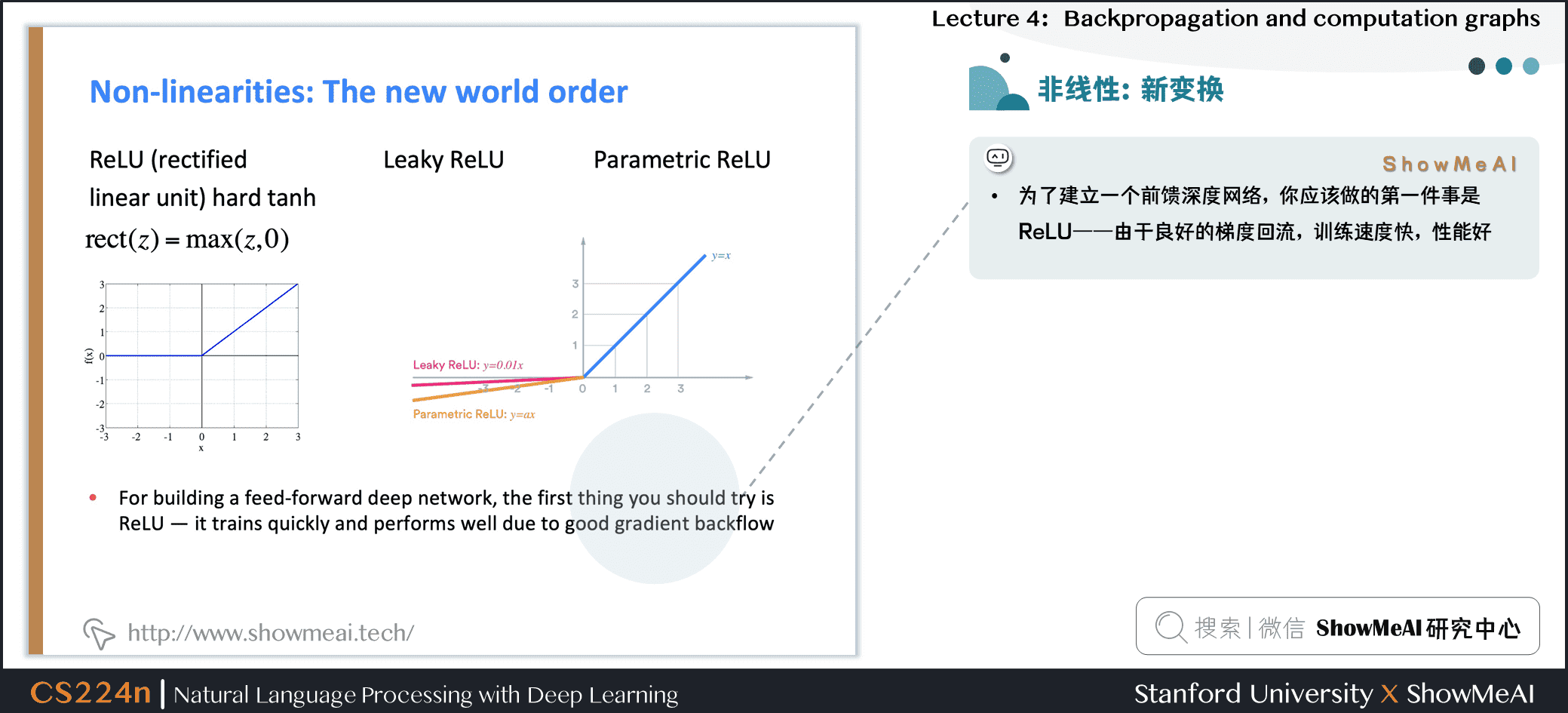• 为了建立一个前馈深度网络，你应该做的第一件事是ReLU——由于良好的梯度回流，训练速度快，性能好

tipReLU

• 每个单元要么已经死了，要么在传递信息。
• 非零范围内只有一个斜率，这一位置梯度十分有效的传递给了输入，所以模型非常有效的训练

## 3.5 参数初始化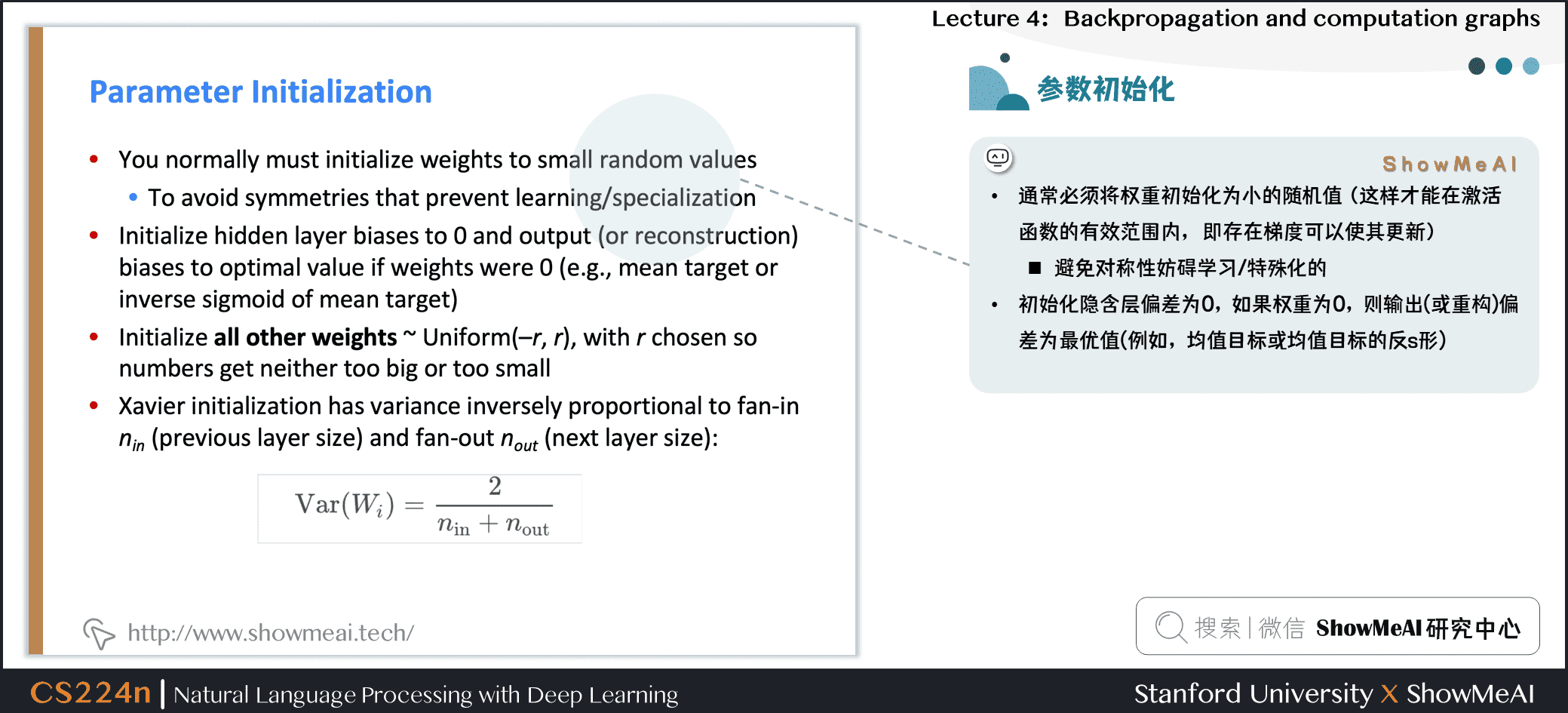• 通常 必须将权重初始化为小的随机值 （这样才能在激活函数的有效范围内， 即存在梯度可以使其更新） 避免对称性妨碍学习/特殊化的
• 初始化隐含层偏差为0，如果权重为0，则输出(或重构)偏差为最优值(例如，均值目标或均值目标的反s形)
• 初始化 所有其他权重为 Uniform(–r, r)，选择使数字既不会太大也不会太小的 r
• Xavier初始化中，方差与 fan-in n_{in} (前一层尺寸)和 fan-out n_{out}(下一层尺寸)成反比
• Var(W_i)=\frac{2}{n_{in}+n_{out}}

## 3.6 优化算法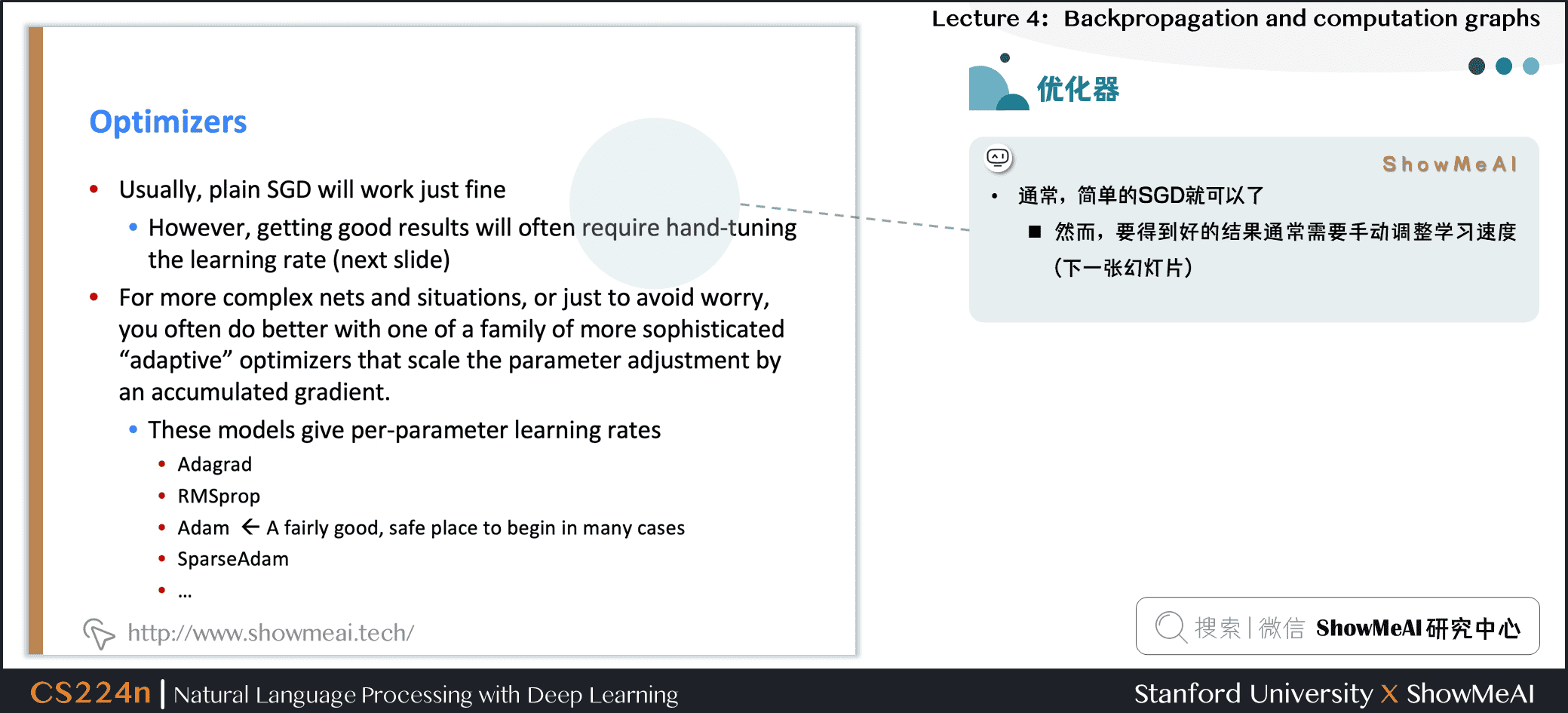通常，简单的SGD就可以了

• 然而，要得到好的结果通常需要手动调整学习速度(下一张幻灯片)
• 对于更复杂的网络和情况，或者只是为了避免担心，更有经验的复杂的 “自适应”优化器通常会令你做得更好，通过累积梯度缩放参数调整。
• RMSprop

## 3.7 学习率策略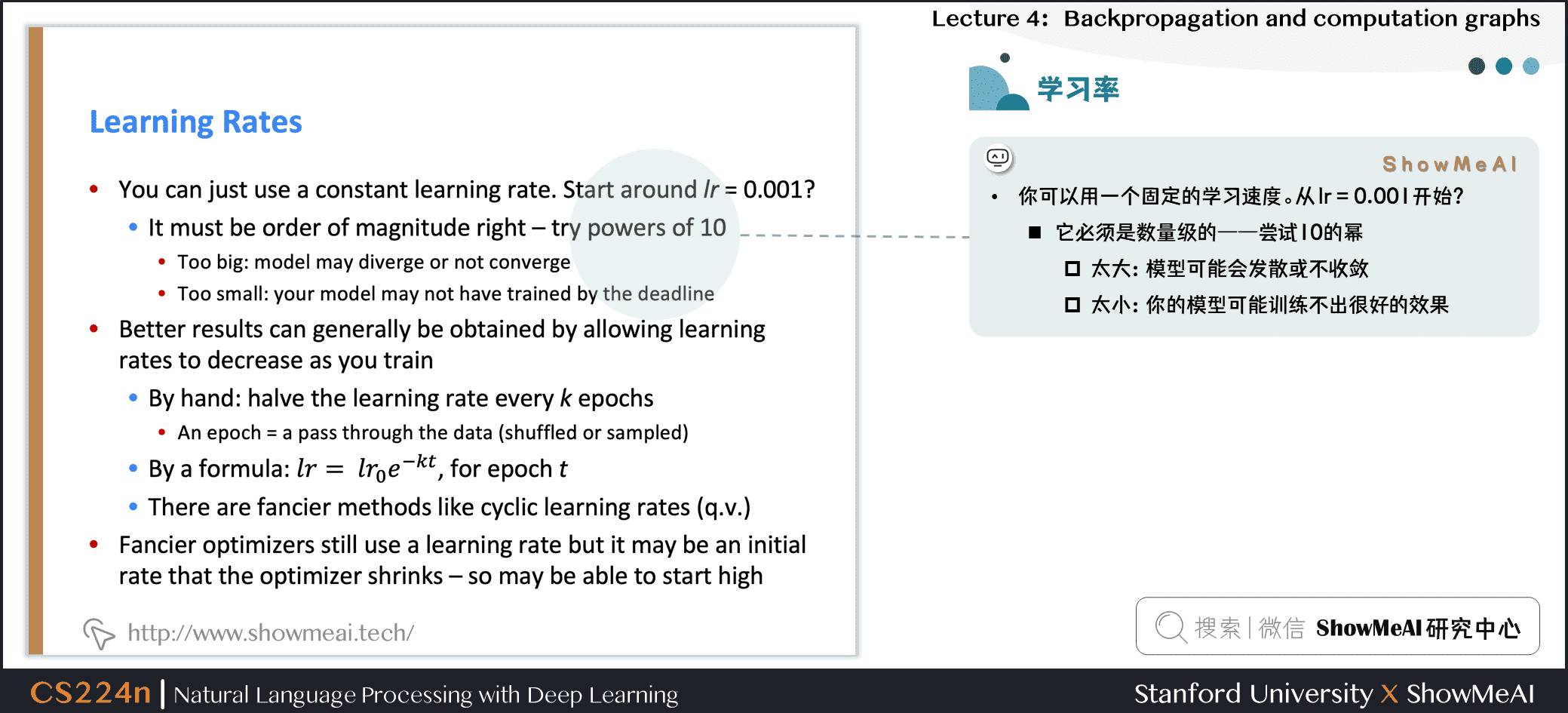• 你可以用一个固定的学习速度。从 $lr = 0.001$开始？

它必须是数量级的——尝试10的幂
• 太大：模型可能会发散或不收敛
• 太小：你的模型可能训练不出很好的效果
• 如果你在训练时降低学习速度，通常可以获得更好的效果

手工：每隔 k 个阶段(epoch)将学习速度减半 epoch = 遍历一次数据 (打乱或采样的)
• 通过一个公式： l r=l r_{0} e^{-k t}, {for epoch }t
• 还有更新奇的方法，比如循环学习率(q.v.)
• 更高级的优化器仍然使用学习率，但它可能是优化器缩小的初始速度——因此可能可以从较高的速度开始

• # 4.视频教程

可以点击 B站 查看视频的【双语字幕】版本

# 斯坦福 CS224n 课程带学详解内容来自用户分享和网络整理，不保证内容的准确性，如有侵权内容，可联系管理员处理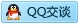标签：
相关文章推荐
新的分享
章节导航